# JP4808907B2 - Multi-carrier CDMA communication apparatus and multi-carrier CDMA transmission apparatus - Google Patents

## Info

Publication number
JP4808907B2
JP4808907B2 JP2002514935A JP2002514935A JP4808907B2 JP 4808907 B2 JP4808907 B2 JP 4808907B2 JP 2002514935 A JP2002514935 A JP 2002514935A JP 2002514935 A JP2002514935 A JP 2002514935A JP 4808907 B2 JP4808907 B2 JP 4808907B2
Authority
JP
Japan
Prior art keywords
subcarrier
frequency
code
Prior art date
Legal status (The legal status is an assumption and is not a legal conclusion. Google has not performed a legal analysis and makes no representation as to the accuracy of the status listed.)
Expired - Fee Related
Application number
JP2002514935A
Other languages
Japanese (ja)
Inventor

Original Assignee

Priority date (The priority date is an assumption and is not a legal conclusion. Google has not performed a legal analysis and makes no representation as to the accuracy of the date listed.)
Filing date
Publication date
Priority to JP2000226027 priority Critical
Priority to JP2000226027 priority
Priority to JP2000259858 priority
Priority to JP2000259858 priority
Application filed by 三菱電機株式会社 filed Critical 三菱電機株式会社
Priority to JP2002514935A priority patent/JP4808907B2/en
Priority to PCT/JP2001/006344 priority patent/WO2002009334A1/en
Application granted granted Critical
Publication of JP4808907B2 publication Critical patent/JP4808907B2/en
Expired - Fee Related legal-status Critical Current
Anticipated expiration legal-status Critical

• 238000004891 communication Methods 0.000 title claims description 82
• 108010003272 hyaluronate lyase Proteins 0.000 claims description 112
• 230000000051 modifying Effects 0.000 claims description 105
• 230000000875 corresponding Effects 0.000 claims description 78
• 230000001360 synchronised Effects 0.000 claims description 73
• 239000000562 conjugates Substances 0.000 claims description 51
• 230000001702 transmitter Effects 0.000 claims description 20
• 238000006243 chemical reaction Methods 0.000 claims description 15
• 238000003786 synthesis Methods 0.000 description 33
• 238000000034 methods Methods 0.000 description 28
• 230000000694 effects Effects 0.000 description 26
• 230000001629 suppression Effects 0.000 description 5
• 238000000354 decomposition Methods 0.000 description 3
• 239000000203 mixtures Substances 0.000 description 2

## Images

••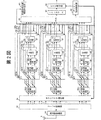•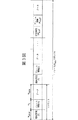•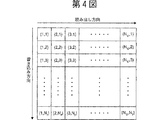•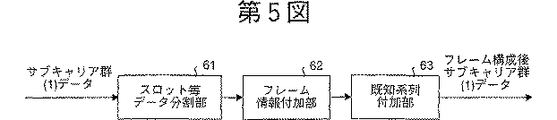•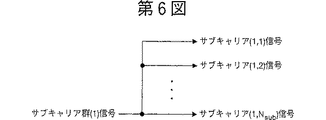•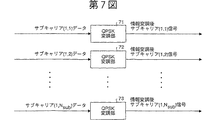•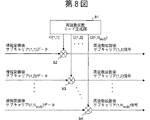•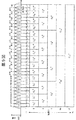•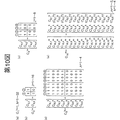•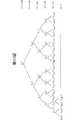•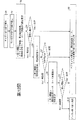•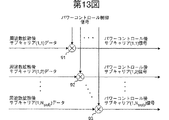•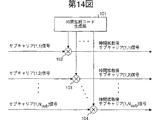•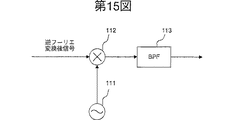•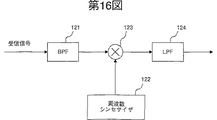•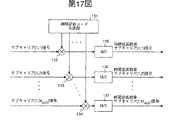•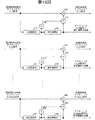•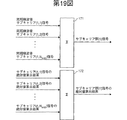•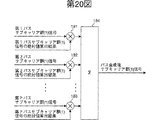•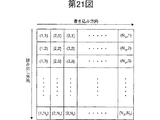•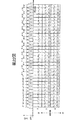•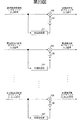•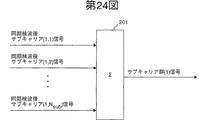•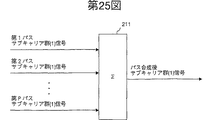••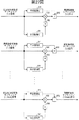•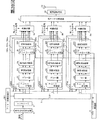•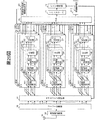•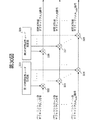•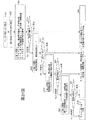•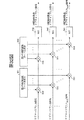•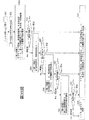•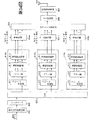•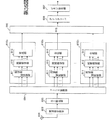•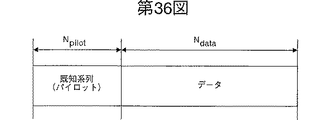•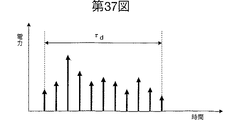••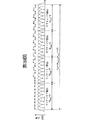## Classifications

• HELECTRICITY
• H04ELECTRIC COMMUNICATION TECHNIQUE
• H04JMULTIPLEX COMMUNICATION
• H04J13/00Code division multiplex systems
• H04J13/16Code allocation
• H04J13/18Allocation of orthogonal codes
• H04J13/20Allocation of orthogonal codes having an orthogonal variable spreading factor [OVSF]
• HELECTRICITY
• H04ELECTRIC COMMUNICATION TECHNIQUE
• H04BTRANSMISSION
• H04B1/00Details of transmission systems, not covered by a single one of groups H04B3/00 - H04B13/00; Details of transmission systems not characterised by the medium used for transmission
• H04B1/692Hybrid techniques using combinations of two or more spread spectrum techniques
• HELECTRICITY
• H04ELECTRIC COMMUNICATION TECHNIQUE
• H04JMULTIPLEX COMMUNICATION
• H04J13/00Code division multiplex systems
• H04J13/0007Code type
• H04J13/004Orthogonal
• H04J13/0044OVSF [orthogonal variable spreading factor]
• HELECTRICITY
• H04ELECTRIC COMMUNICATION TECHNIQUE
• H04LTRANSMISSION OF DIGITAL INFORMATION, e.g. TELEGRAPHIC COMMUNICATION
• H04L5/00Arrangements affording multiple use of the transmission path
• H04L5/02Channels characterised by the type of signal
• H04L5/023Multiplexing of multicarrier modulation signals
• H04L5/026Multiplexing of multicarrier modulation signals using code division
• HELECTRICITY
• H04ELECTRIC COMMUNICATION TECHNIQUE
• H04BTRANSMISSION
• H04B1/00Details of transmission systems, not covered by a single one of groups H04B3/00 - H04B13/00; Details of transmission systems not characterised by the medium used for transmission
• H04B1/707Spread spectrum techniques using direct sequence modulation
• HELECTRICITY
• H04ELECTRIC COMMUNICATION TECHNIQUE
• H04BTRANSMISSION
• H04B1/00Details of transmission systems, not covered by a single one of groups H04B3/00 - H04B13/00; Details of transmission systems not characterised by the medium used for transmission
• H04B1/707Spread spectrum techniques using direct sequence modulation
• H04B1/7097Interference-related aspects
• H04B1/711Interference-related aspects the interference being multi-path interference
• H04B1/7115Constructive combining of multi-path signals, i.e. RAKE receivers
• H04B1/712Weighting of fingers for combining, e.g. amplitude control or phase rotation using an inner loop

## Description

Technical field
The present invention relates to a communication apparatus of a mobile communication system that employs a multiple access method using a multicarrier CDMA method, and particularly, excellent bit error rate characteristics even in a communication environment affected by frequency selective fading. The present invention relates to a multicarrier CDMA communication apparatus, a multicarrier CDMA transmission apparatus, and a multicarrier CDMA reception apparatus.
Background art
A conventional multi-carrier CDMA communication apparatus will be described below. As a communication apparatus of a mobile communication system adopting a multiple access method using a multicarrier CDMA method, for example, the document “SC / DS-CDMA, MC / DS-CDMA, MC-CDMA method in downlink broadband wireless packet transmission” Characteristics comparison, IEICE Technical Report RCS99-130 p.63-70 October 1999, "Overview of Multicarrier CDMA, IEEE Communications Magazine p.126-133 December 1997" There is.
Here, the configuration and operation of the conventional multicarrier CDMA communication apparatus will be described with reference to the drawings. FIG. 34 is a diagram showing the configuration of a conventional multicarrier CDMA transmitter (hereinafter simply referred to as a transmitter), and FIG. 35 is a conventional multicarrier CDMA receiver (hereinafter simply referred to as a receiver). FIG.
In FIG. 34, 501 is a convolutional coding unit, 502 is an interleaver, 503 is a serial / parallel conversion unit (hereinafter referred to as S / P), and 510a, 510b, and 510c are the first, second, An Nscg-th subcarrier group modulation processing unit, 511 is a frame creation unit, 512 is a copy unit, 513 is an information modulation unit, 514 is a frequency spreading unit, and 504a, 504b, and 504c are multiplexed. 505 is an inverse Fourier transform unit, 506 is a guard interval (GI) addition unit, 507 is a frequency conversion unit, and 508 is an antenna.
On the other hand, in FIG. 35, 601 is an antenna, 602 is a frequency conversion unit, 603 is a guard interval (GI) removal unit, 604 is a Fourier transform unit, and 610a, 610b, and 610c are 1, 2, Nscg-th subcarrier group demodulation processing unit, 611 is a frequency despreading unit, 612 is a synchronous detection unit, 613 is a synthesis unit, and 605 is a parallel / serial conversion unit (hereinafter, 606 is a deinterleaver unit, and 607 is a Viterbi decoding unit.
FIG. 36 shows the format of a transmission slot in units of subcarriers. Thus, the transmission slot is composed of a pilot symbol part (known sequence) and a data part.
FIG. 37 is a diagram showing an example of the impulse response of the frequency selective fading transmission line. For example, in mobile communication systems, radio waves are reflected, diffracted, and scattered by surrounding buildings and terrain, and arriving waves (multipath waves) that have passed through multiple transmission paths interfere with each other. An impulse response of the fading transmission line occurs.
The operation of the conventional multi-carrier CDMA communication apparatus will be described below with reference to FIGS. 34 and 35. Here, data transmission / reception by a base station and a plurality of terminals is assumed. First, the operation of the transmission apparatus will be described.
For example, the convolutional encoding unit 501 that has received the transmission data for a given terminal generates encoded data according to a predetermined encoding rate. This encoded data is written in the vertical direction with respect to the interleaver 502 including, for example, a block having a vertical size of Nr (predetermined integer) and a horizontal size of Nc (predetermined integer). Read out horizontally. That is, interleaver 502 outputs the rearranged signal as encoded data.
In the S / P 503 that has received the encoded data, the data is converted into Nscg (predetermined integer) parallel data, and the converted outputs are sent to the subcarrier group modulation processing units 510a, 510b,. Output. In the first to Nscg-th subcarrier group modulation processing units that perform modulation processing in units of subcarrier groups, the same signal processing is performed, so here the first subcarrier group modulation processing unit Only the operation of 510a will be described, and description of other subcarrier group modulation processing units will be omitted.
Multiplexer 504a multiplexes signals for the same number of subcarriers from other users with respect to the received signals for the number of subcarriers after frequency spreading to generate a multiplexed signal.
The inverse Fourier transform unit 505 performs an inverse Fourier transform process using the Nsgg × Nsub subcarrier signals obtained by the multiplexing units 504a, 504b, and 504c.
In the guard interval adding unit 506, the rear part of the symbol in the signal after the inverse Fourier transform is τGICopy only the amount of time, and paste the copy at the beginning of the symbol. FIG. 38 is a diagram showing processing of the guard interval adding unit 506. ΤGIIs generally delayed wave spread τ on the transmission line shown in FIG.dIt is set to be larger.
Finally, the frequency conversion unit 507 multiplies the signal after the addition of the guard interval by a carrier wave signal output from a frequency oscillator (not shown), and further limits the band using a span path filter (not shown). By performing this, a transmission signal is generated, and then the transmission signal is output on the transmission path via the antenna 508. FIG. 39 is a diagram showing a transmission signal expressed on the frequency axis.
On the other hand, the receiving apparatus receives a transmission signal affected by frequency selective fading or the like via the antenna 601, and the frequency converter 602 performs band limitation on the received signal by a bandpass filter (not shown). Then, the signal after the band limitation is multiplied by a signal synchronized with the carrier frequency output by a frequency synthesizer (not shown). Further, the low-frequency component of the multiplied signal is filtered by a low-pass filter (not shown), and the resultant signal is output as a signal after frequency conversion.
A guard interval (GI) removal unit 603 removes the guard interval and outputs a signal continuously connected in symbol units. Upon receiving the signal after removal of the guard interval, the Fourier transform unit 604 performs Fourier transform processing and outputs Nsgg × Nsub subcarrier signals. Each subcarrier signal is transmitted to the first, second,..., Nscg-th subcarrier group demodulation processing units 610a, 610b, and 610c in order to perform demodulation processing in units of subcarrier groups. Since the first, second, and Nscg subcarrier group demodulation processing units 610a, 610b, and 610c perform the same signal processing, only the operation of the first subcarrier group demodulation processing unit 610a is performed here. The description will be made, and description of other subcarrier group demodulation processing units will be omitted.
Subcarrier group demodulation processing section 610a receives the first Nsub subcarrier signals. First, frequency despreading section 611 multiplies Nsub subcarrier signals by individually assigned spreading codes. By doing so, despreading processing is performed.
The synchronous detector 612 that has received each subcarrier signal after frequency despreading estimates the transmission path using the known sequence symbol added in frame units, and performs synchronous detection. That is, synchronous detection section 612 first calculates a transmission path estimation value for each subcarrier by synchronously adding Npilot known sequence symbols in a frame. Next, a complex conjugate value and an absolute value of the calculation result are calculated, and further, the phase component is extracted in subcarrier units by dividing the complex conjugate value by the absolute value. Finally, synchronous detection is performed by multiplying the subcarrier signal after frequency despreading by a phase component in units of subcarriers.
The synthesizer 613 adds all the received subcarrier signals after synchronous detection to calculate the first subcarrier group signal.
In P / S605, subcarrier group signals are received from all subcarrier group demodulation processing units, and converted into serial signals. The serial signal is written in the horizontal direction to a deinterleaver 606 including a block having a vertical size of Nr (predetermined integer) and a horizontal size of Nc (predetermined integer). Read out vertically.
Finally, the Viterbi decoding unit 607 performs known Viterbi decoding on the received rearranged signals.
As described above, in the conventional multicarrier CDMA communication apparatus, even when the received wave is affected by frequency selective fading in which the amplitude and phase of the received wave fluctuate randomly, for example, guarding is performed so that the spread of the delayed wave is reduced. A good bit error rate characteristic has been obtained by setting an interval and assigning a frequency spreading code to each user or channel.
However, in the above-described conventional multicarrier CDMA communication apparatus, there are cases where the spread of the delayed wave is large depending on the transmission path condition and does not fit within the guard interval. In such a case, there has been a problem that the apparatus is affected by frequency selective fading, and the influence of interference within a symbol becomes large, and a good bit error rate characteristic cannot be obtained.
Further, in the conventional multicarrier CDMA communication apparatus, even when the level of the delayed wave is higher than that of the preceding wave, the delayed wave has the received signal quality (for example, signal power to interference power ratio (SIR)). There was a problem that it was not used to increase it.
In multimedia mobile communication, it is considered necessary to adaptively change the information speed depending on the application to be handled. In other words, when assigning the frequency spreading code to the user unit or the channel unit to be used, it is necessary to assign the channel according to the information transmission rate. However, in the conventional multi-carrier CDMA communication apparatus, the frequency spreading codes need to be orthogonal to each other and the frequency spreading factor is fixed, so that the information rate cannot be adaptively changed. there were.
Further, in the conventional multicarrier CDMA communication apparatus, when performing handover between sectors and between cells so that communication is not interrupted when a mobile station (terminal) is received, it is necessary to change the frequency used on the transmission path. Therefore, there is a problem that the frequency use efficiency is reduced.
Also, in the conventional multicarrier CDMA communication apparatus, transmission power control is required on the base station side in order to solve the problem that the reception quality of the mobile station must be kept constant regardless of the distance from the base station. However, in land mobile communication, the influence of frequency selective fading is greatly different for each subcarrier. Therefore, the conventional method of keeping the received signal power constant at the mobile station has a problem that the received signal quality cannot be kept constant while suppressing the influence on other mobile stations (terminals).
Therefore, the present invention can realize a good bit error rate characteristic even when the spread of the delayed wave does not fall within the guard interval and the transmission signal from the base station is affected by frequency selective fading on the transmission line. An object of the present invention is to provide a multi-carrier CDMA communication apparatus.
It is another object of the present invention to provide a multi-carrier CDMA communication apparatus that can improve the quality of a received signal using a delayed wave when the level of the delayed wave is higher than that of a preceding wave. Yes.
It is another object of the present invention to provide a multi-carrier CDMA communication apparatus capable of changing a frequency spreading factor while maintaining a state where frequency spreading codes assigned to a user unit or a channel unit to be used are orthogonal to each other.
In addition, the present invention provides a multicarrier CDMA communication apparatus capable of executing soft handover between sectors or cells without interrupting communication and changing the frequency used on the transmission path at the time of reception. It is aimed.
Further, the present invention reduces received signal quality while suppressing the influence on other mobile stations (terminals) even when the influence of frequency selective fading is greatly different for each subcarrier, that is, while reducing the amount of interference. It is an object of the present invention to provide a multicarrier CDMA communication apparatus capable of keeping the frequency constant.
Disclosure of the invention
In the multicarrier CDMA communication apparatus according to the present invention, the transmission side performs frequency spreading processing for each subcarrier signal unit of each channel constituting the subcarrier group based on a predetermined condition. Signal modulation means (corresponding to the subcarrier group modulation processing units 6a to 8c), and all signals after the modulation processing and the frequency spread processing are multiplexed in units of subcarrier signals, and multiplexed signals in units of the subcarrier signals are obtained. Time spreading means (corresponding to multiplexing units 9a to 9c and time spreading units 10a to 10c) corresponding to the number of subcarrier groups for performing time spreading processing on the receiving side, and the receiving side performs time despreading in units of subcarrier signals Time despreading means (corresponding to time despreading units 35a to 35c) corresponding to the number of subcarrier groups for processing, and subcarrier signal units after the time despreading processing Performing wavenumber despreading, a subcarrier number of a signal demodulating means group (corresponding to a sub-carrier group demodulation processing section 36A～38c), characterized in that it comprises a.
In the multicarrier CDMA communication apparatus according to the next invention, the signal modulation means creates a frame for creating a data frame composed of a known sequence, frame information, and data for each subcarrier group based on the condition. A frame creation unit 21), a copy means for copying the data frame and generating data frames for the number of subcarriers (equivalent to the copy unit 22), and information modulation for performing a modulation process on each data frame Means (corresponding to the information modulating unit 23), frequency spreading means (corresponding to the frequency spreading unit 24) for performing frequency spreading processing on each of the modulated subcarrier signals based on the conditions, and the frequency spreading processing Power control means (corresponding to the power control unit 25) for performing transmission power control on each subsequent subcarrier signal Characterized in that it comprises a and.
In the multicarrier CDMA communication apparatus according to the next invention, the setting means sets the error correction coding rate based on a received signal power-to-interference power ratio sent from the receiving side, and further, the frequency When setting the spreading code, if there is no frequency spreading code to be assigned, the assignable frequency spreading code is secured by lowering the frequency spreading factor.
In the multicarrier CDMA communication apparatus according to the next invention, the setting means sets the error correction coding rate based on a received signal power-to-interference power ratio sent from the receiving side, and further, the frequency If there is no frequency spreading code to be assigned when setting the spreading code, an assignable frequency spreading code is secured by lowering the frequency spreading factor. If setting is impossible even in this state, within the same subcarrier group By securing a plurality of frequency spreading codes, an assignable frequency spreading code is secured.
In the multi-carrier CDMA communication apparatus according to the next invention, the setting means further assigns the frequency spreading code at a predetermined frequency interval while maintaining the orthogonality and hierarchical relationship between the frequency spreading codes. To do.
In the multicarrier CDMA communication apparatus according to the next invention, the signal demodulating means is added to a data frame and frequency despreading means (corresponding to the frequency despreading section 54) for performing frequency despreading processing on a subcarrier signal basis. Based on the known sequence, the transmission path is estimated for each subcarrier signal after frequency inverse transform, and the absolute value and the complex conjugate value of the transmission path estimation result are calculated, and then the complex conjugate value is calculated by the absolute value. Synchronous detection means (corresponding to the synchronous detection unit 55) that normalizes, weights the subcarrier signal with the normalization result, and outputs the absolute value and the weighted subcarrier signal as an output; All subcarrier signals are added to generate a signal as the subcarrier group, and all the absolute values are added to the subcarrier. And a means for generating an absolute value (corresponding to the combining unit 56), a subcarrier group signal corresponding to each path is multiplied by the absolute value of the corresponding subcarrier group, and all the multiplication results are added. Path synthesis means (corresponding to the path synthesis unit 57) for generating a subcarrier group signal after path synthesis.
In the multicarrier CDMA communication apparatus according to the next invention, the signal demodulating means includes frequency despreading means for performing frequency despreading processing on the subcarrier signal unit, and frequency inverse transform based on a known sequence added to the data frame. Transmission path estimation is performed for each subsequent subcarrier signal unit, a complex conjugate value of the transmission path estimation result is calculated, then the subcarrier signal is weighted by the complex conjugate value, and the weighted subcarrier is output as an output. Synchronous detection means for outputting a signal, combining means for adding all the weighted subcarrier signals to generate a signal as the subcarrier group, and adding all subcarrier group signals corresponding to each path Path combining means for generating a combined subcarrier group signal.
In the multicarrier CDMA communication apparatus according to the next invention, the signal demodulating means includes frequency despreading means for performing frequency despreading processing on the subcarrier signal unit, and frequency inverse transform based on a known sequence added to the data frame. The transmission path is estimated for each subsequent subcarrier signal unit, the absolute value and the complex conjugate value of the transmission path estimation result are calculated, then the complex conjugate value is normalized by the absolute value, and the subcarrier signal is Synchronous detection means for weighting according to a normalization result, on the other hand, estimating interference power in a subcarrier signal unit after frequency inverse transform based on the known sequence, and dividing the weighted subcarrier signal by the interference power; All subcarrier signals after synchronous detection are added to generate a signal as the subcarrier group, and all the absolute values are The combination means for generating an absolute value as the subcarrier group by multiplying the subcarrier group signal corresponding to each path by the absolute value of the corresponding subcarrier group, and adding all the multiplication results Path synthesis means for generating a subcarrier group signal after path synthesis.
In the multicarrier CDMA communication apparatus according to the next invention, the signal demodulating means includes frequency despreading means for performing frequency despreading processing on the subcarrier signal unit, and frequency inverse transform based on a known sequence added to the data frame. Perform transmission path estimation for each subsequent subcarrier signal, calculate a complex conjugate value of the transmission path estimation result, then weight the subcarrier signal with the complex conjugate value, and finally, the weighted subcarrier signal Synchronous detection means for removing interference components from the signal, combining means for adding all the subcarrier signals after the interference removal to generate a signal as the subcarrier group, and adding all subcarrier group signals corresponding to each path And path synthesis means for generating a subcarrier group signal after path synthesis.
In the multicarrier CDMA communication apparatus according to the next invention, the transmission side performs frequency spreading processing and time spreading processing on the subcarrier signal unit of each channel constituting the subcarrier group based on a predetermined condition. Subcarriers that include signal modulation means (corresponding to subcarrier group modulation processing units 282a to 284c) for the number of subcarrier groups, and the receiving side performs time despreading processing and frequency despreading processing for each subcarrier signal. It comprises signal demodulating means (corresponding to subcarrier group demodulation processing sections 301a to 303c) for the number of groups.
In the multi-carrier CDMA communication apparatus according to the next invention, the transmission side has an error correction coding rate, the number of subcarrier groups, a frequency spreading factor, a code multiplexing number of a frequency spreading code, a frequency spreading code, a time spreading factor, Setting means (corresponding to the data / modulation control unit 281) for setting the number of multiplexed code of the time spreading code and the time spreading code, and, based on the setting, for each subcarrier signal unit of each channel constituting the subcarrier group And signal modulation means (corresponding to subcarrier group modulation processing units 282a to 284c) for the number of subcarrier groups that perform frequency spreading processing and time spreading processing, and the receiving side performs time despreading in units of subcarrier signals. Signal demodulating means (subcarrier group demodulation processing sections 301a to 303c) corresponding to the number of subcarrier groups for performing processing and frequency despreading processing. ), Characterized in that it comprises a.
In the multicarrier CDMA communication apparatus according to the next invention, the signal modulation means includes a frame creation means for creating a data frame composed of a known sequence, frame information, and data for each subcarrier group based on the condition. A copy means for copying the data frame and generating data frames for the number of subcarriers; an information modulation means for performing a modulation process on each data frame; and each subframe after the modulation based on the condition Frequency spreading means for performing frequency spreading processing on a carrier signal, power control means for performing transmission power control on each subcarrier signal after the frequency spreading processing, and for each subcarrier signal after the frequency spreading processing And a time diffusion means (corresponding to the time diffusion unit 291) for performing time diffusion processing. That.
In the multicarrier CDMA communication apparatus according to the next invention, the setting means sets the error correction coding rate based on a received signal power-to-interference power ratio sent from the receiving side, and further, the frequency When setting the spreading code and the time spreading code, if there is no frequency spreading code and time spreading code to be assigned, the assignable frequency spreading code is secured by lowering the frequency spreading factor.
In the multicarrier CDMA communication apparatus according to the next invention, the signal demodulating means includes time despreading means (corresponding to the time despreading section 311) for performing time despreading processing on the subcarrier signal unit, and the subcarrier signal unit. And frequency despreading means for performing frequency despreading processing, and channel estimation for each subcarrier signal after frequency deconversion based on a known sequence added to the data frame, and the absolute value of the channel estimation result and the complex Calculate the conjugate value, then normalize the complex conjugate value by the absolute value, weight the subcarrier signal by the normalization result, and output the absolute value and the weighted subcarrier signal as output And a synchronous detection means for adding all the weighted subcarrier signals to generate a signal as the subcarrier group. A combination means for adding all the absolute values to generate an absolute value as the subcarrier group, and multiplying the subcarrier group signal corresponding to each path by the absolute value of the corresponding subcarrier group, Path synthesis means for adding all and generating a subcarrier group signal after path synthesis.
In the multicarrier CDMA communication apparatus according to the next invention, the signal demodulating means includes time despreading means for performing time despreading processing for each subcarrier signal unit, and frequency despreading for performing frequency despreading processing for each subcarrier signal unit. Based on a spreading means and a known sequence added to the data frame, transmission path estimation is performed in units of subcarrier signals after frequency inverse transform, a complex conjugate value of the transmission path estimation result is calculated, and then the subcarrier signal Is combined with the complex conjugate value to generate a signal as the subcarrier group by adding the synchronous detection means for outputting the weighted subcarrier signal as an output, and adding all the weighted subcarrier signals. And subcarrier group signals corresponding to each path are added together to generate a subcarrier group signal after path synthesis. Characterized in that it comprises a path combining means.
In the multicarrier CDMA communication apparatus according to the next invention, the signal demodulating means includes time despreading means for performing time despreading processing for each subcarrier signal unit, and frequency despreading for performing frequency despreading processing for each subcarrier signal unit. Based on the spreading means and the known sequence added to the data frame, performs transmission path estimation in units of subcarrier signals after frequency inverse transform, calculates the absolute value and complex conjugate value of the transmission path estimation result, and then The complex conjugate value is normalized by the absolute value, the subcarrier signal is weighted by the normalization result, and the interference power is estimated for each subcarrier signal unit after frequency inverse transform based on the known sequence, and weighted Synchronous detection means that divides the subsequent subcarrier signal by the interference power, and all the subcarrier signals after the synchronous detection are added together. A combining unit that generates a signal as a subcarrier group and further adds all the absolute values to generate an absolute value as the subcarrier group, and a subcarrier group signal corresponding to each path. Path synthesis means for multiplying the absolute value of the carrier group and adding all the multiplication results to generate a subcarrier group signal after path synthesis.
In the multicarrier CDMA communication apparatus according to the next invention, the signal demodulating means includes time despreading means for performing time despreading processing for each subcarrier signal unit, and frequency despreading for performing frequency despreading processing for each subcarrier signal unit. Based on a spreading means and a known sequence added to the data frame, transmission path estimation is performed in units of subcarrier signals after frequency inverse transform, a complex conjugate value of the transmission path estimation result is calculated, and then the subcarrier signal Synchronous detection means for estimating the interference power in units of subcarrier signals after frequency inverse transform based on the known sequence, and dividing the weighted subcarrier signal by the interference power, Combining means for adding all the subcarrier signals after the synchronous detection to generate signals as the subcarrier group; and And path combining means for adding all the sub-carrier group signal to generate a sub-carrier group signal after path combining corresponding to the scan, characterized in that it comprises a.
In the multicarrier CDMA transmitting apparatus according to the next invention, signals corresponding to the number of subcarrier groups that perform frequency spreading processing on a subcarrier signal basis of each channel constituting the subcarrier group based on a predetermined condition. A time corresponding to the number of subcarrier groups, which multiplexes all the signals after the modulation means and the modulation processing and the frequency spread processing in units of subcarrier signals and performs time spread processing on the multiplexed signals in units of the subcarrier signals. And a diffusing unit.
In the multi-carrier CDMA transmitter according to the next invention, the error correction coding rate, the number of subcarrier groups, the frequency spreading factor, the number of code multiplexes of the frequency spreading code, the frequency spreading code, the time spreading factor, and the time spreading code Setting means for setting the number of code multiplexes and a time spreading code, and frequency spreading processing is performed for each subcarrier signal unit of each channel constituting the subcarrier group based on the setting condition. Signal modulation means, and all signals after the modulation process and the frequency spread process are multiplexed in units of subcarrier signals, and time spread processing is performed on the multiplexed signals in units of the subcarrier signals. And a time spreading means.
In the multicarrier CDMA transmitter according to the next invention, the subcarrier group performs frequency spreading processing and time spreading processing for each subcarrier signal unit of each channel constituting the subcarrier group based on a predetermined condition. And several minutes of signal modulation means.
In the multi-carrier CDMA transmitter according to the next invention, the error correction coding rate, the number of subcarrier groups, the frequency spreading factor, the number of code multiplexes of the frequency spreading code, the frequency spreading code, the time spreading factor, and the time spreading code A setting means for setting the number of code multiplexes and a time spreading code, and a sub-carrier signal unit for each channel constituting a sub-carrier group, which performs frequency spreading processing and time spreading processing based on the setting conditions. Signal modulation means for the number of carrier groups.
In the multicarrier CDMA receiver according to the next invention, time despreading means for the number of subcarrier groups for performing time despreading processing for each subcarrier signal, and subcarrier signal units after the time despreading processing And a signal demodulating unit for performing the frequency despreading processing for the number of subcarrier groups.
The multicarrier CDMA receiver according to the next invention is characterized by comprising signal demodulating means for the number of subcarrier groups for performing time despreading processing and frequency despreading processing for each subcarrier signal. .
BEST MODE FOR CARRYING OUT THE INVENTION
Embodiments of a multicarrier CDMA communication apparatus according to the present invention will be described below in detail with reference to the drawings. Note that the present invention is not limited to the embodiments.
Embodiment 1 FIG.
1 and 2 are diagrams showing a configuration of a first embodiment of a multicarrier CDMA communication apparatus according to the present invention. In detail, FIG. 1 shows a multicarrier CDMA transmission apparatus according to the present embodiment. FIG. 2 is a diagram showing a configuration of a multicarrier CDMA receiving apparatus (hereinafter simply referred to as a receiving apparatus) of the present embodiment.
In FIG. 1, 1 is a convolutional coding unit, 2 is an interleaver, 3 is a serial / parallel conversion unit (hereinafter referred to as S / P), 4 is a data / modulation control unit, and 5 Is a power control control unit, and 6a, 6b, 6c, 7a, 7b, 7c,..., 8a, 8b, and 8c are first, second, and Nscg subcarrier group modulation processing units provided for each channel. 9a, 9b, 9c are multiplexing units, 10a, 10b, 10c are time spreading units, 11 is an inverse Fourier transform unit, 12 is a frequency conversion unit, 13 is an antenna, 21 Is a frame creation unit, 22 is a copy unit, 23 is an information modulation unit, 24 is a frequency spreading unit, and 25 is a power control unit.
On the other hand, in FIG. 2, 31 is an antenna, 32 is a frequency conversion unit, 33 is a Fourier transform unit, 34 is a subcarrier selection unit, and 35a, 35b, and 35c are the first and second units, respectively. , Nscg-th subcarrier group time despreading unit, and 36a, 36b, 36c, 37a, 37b, 37c, ..., 38a, 38b, 38c are the first, second, Nscg-th provided for each multiplexed channel. , 39 is a parallel / serial converter (P / S), 40 is a received signal quality information generator, 41 is a deinterleaver, and 42 is a Viterbi decoder. , 43 is a data / demodulation control unit, 51, 52 and 53 are demodulation processing units for each path, 54 is a frequency despreading unit, 55 is a synchronous detection unit, and 56 is a synthesis unit. , 57 is the path combining section.
FIG. 3 is a diagram showing a format of a transmission slot in units of subcarriers. Thus, the transmission slot is composed of a pilot symbol part (known sequence), a frame information part, and a data part.
Hereinafter, operations of the transmission apparatus and the reception apparatus according to the present embodiment will be described in detail with reference to FIGS. Here, data transmission / reception by a base station and a plurality of terminals is assumed. First, the operation of the transmission apparatus will be described.
First, the convolutional encoding unit 1 that has received transmission data for an arbitrary terminal sends a “code” for setting an error correction encoding rate according to desired communication quality sent from the data / modulation control unit 4. The transmission signal is convolutionally coded on the basis of the “configuration ratio setting signal”.
The interleaver 2 rearranges the convolutionally encoded data. FIG. 4 is a diagram showing the configuration of the interleaver 2. In the present embodiment, for example, a block having a vertical size of Nr (predetermined integer) and a horizontal size of Nc (predetermined integer) is provided. By writing in the vertical direction and then reading in the horizontal direction, the encoded data is rearranged in units of frames.
In S / P3, the rearranged encoded data and the number of subcarrier groups Nsgg, frequency spreading factor Nsub, and code multiplexing number M on the frequency axis sent from the data / modulation control unit 4 are set. To receive a “setting signal”. The number of subcarrier groups Nscg and the frequency spreading factor Nsub take values of 1 or more and the number of all subcarriers on the frequency band. In S / P3, the encoded data (serial signal) after rearrangement is converted into parallel signals corresponding to all channels of each subcarrier group based on the setting signal.
The subcarrier group modulation processing units 6a to 8c individually corresponding to the M number of code multiplexing numbers of each subcarrier group receive the parallel signal and the setting signal and perform modulation processing on a channel basis. The M subcarrier group modulation processing units 6a to 8c in the first to Nscg subcarrier groups perform the same signal processing, respectively. Here, in the first subcarrier group, Only the operation of the subcarrier group modulation processing unit 6a of the first channel will be described, and description of the other subcarrier group modulation processing units will be omitted. Further, the number Nscg of subcarrier groups and the number M of code multiplexing for each subcarrier group are variables determined by the data / modulation control unit 4.
FIG. 6 is a diagram showing the configuration of the copy unit 22. The copy unit 22 copies the received data frame by the number of subcarriers Nsub in accordance with the frequency spreading factor setting signal output from the data / modulation control unit 4 and outputs subcarrier signals (1, 1) to (1). , Nsub) data frames are generated. Thereafter, these data frames are output to the information modulation unit 23.
FIG. 7 is a diagram showing a configuration of the information modulation unit 23. In FIG. 7, reference numerals 71, 72, 73 denote QPSK modulators. Each QPSK modulator performs QPSK modulation on the received frame data for the number of subcarriers, and generates modulated signals for subcarriers (1, 1) to (1, Nsub). Thereafter, these modulated signals are output to the frequency spreading unit 24.
The frequency spreading code is expressed by a Hadamard-Walsch code, which is one of orthogonal codes. When generalized, the method of generating the frequency spreading code can be expressed by the following equation (1).
When this frequency spreading code is used at the time of frequency spreading, each element is converted from 1 → + 1, 0 → −1, and then the information-modulated subcarrier signal is multiplied. As is clear from the relationship shown in Equation (1), it can be seen that the spread spectrum code has a hierarchical orthogonal relationship under specific constraints.
FIG. 11 is a diagram showing a state in which the frequency spreading codes are hierarchically orthogonal. Here, by using a frequency spreading code that has a hierarchical orthogonal condition as a frequency spreading code, data of a channel having a plurality of frequency spreading factors is transmitted under the specific constraint conditions shown in FIG. be able to. Further, when the frequency spreading factor is reduced, the number of subcarrier groups increases, so that the transmission data rate can be increased.
FIG. 12 is a flowchart showing a method of assigning frequency spreading codes in the data / modulation control unit 4. For example, in a mobile station, in order to maintain the required quality of transmission data, “received signal quality information” generated using a received signal power to interference power ratio (SIR) at the time of reception is generated in a frame transmitted to the base station. insert. This received signal quality information is for making a channel assignment request. Therefore, the data / modulation control unit 4 that has received a channel allocation request for securing a channel of transmission data from the mobile station (step S1) allocates a coding rate based on the received signal quality information (step S2). That is, based on the received signal quality information, a coding rate for error correction using a convolutional code is set. Note that, based on the received signal quality information, the coding rate is set small when the mobile station reception state is bad, and the coding rate is set large when the reception state is good.
Thereafter, the data / modulation control unit 4 assigns the same number of subcarrier groups as the reciprocal of the coding rate as the first stage (step S3). For example, when the coding rate is 1/2 and the frequency spreading factor is 4, the frequency spreading code C in FIG.4 1, C4 2, ..., C4 8A total of two frequency spreading codes are selected from each of the two frequency spreading code groups in the eight frequency spreading code groups. Note that the frequency spreading code to be selected is not the same as the frequency spreading code already occupied by other users and channels. At this time, if the frequency spreading code can be secured (Yes in step S4), the data / modulation control unit 4 determines that the setting is possible, so that the number of subcarrier groups, the number of multiplexed codes, and the allocation of the frequency spreading code are determined ( Step S5).
On the other hand, if the frequency spread code cannot be secured by the setting of the first stage (step S4, No), the data / modulation control unit 4 sets the frequency spread code while maintaining the transmission speed of the transmission data as the second stage. The frequency spreading factor is reset in order to allocate and increase the number of available subcarrier groups. Here, by changing the frequency spreading factor of one or a plurality of subcarrier groups set to 4 to 2 and doubling the number of subcarrier groups that can be used in the same band, the assignable frequency spreading code Is secured (step S6). At this time, if the frequency spreading code can be secured (Yes in step S7), the data / modulation control unit 4 determines that the setting is possible, so that the number of subcarrier groups, the number of code multiplexes, and the frequency spreading code are determined ( Step S5).
Furthermore, when the frequency spreading code cannot be secured by the setting of the second stage (No in step S7), the data / modulation control unit 4 performs the frequency within the same subcarrier group having a frequency spreading factor of 4 as the third stage. A plurality of spreading codes are allowed to be reserved, and a frequency spreading code for multiplexing within the same subcarrier group is set (step S8). At this time, if the frequency spreading code can be secured (Yes in step S9), the data / modulation control unit 4 determines that the setting is possible, so that the number of subcarrier groups, the number of code multiplexes, and the frequency spreading code are determined ( Step S5). On the other hand, if the frequency spreading code cannot be secured (No in step S9), the data / modulation control unit 4 fails in channel assignment (step S10). In the frequency spreading code assignment method, the code multiplexing number is set to 1 at the first stage, but the code multiplexing number may be set to 1 or more from the first stage.
The frequency spreading code set as described above is transmitted as frame information (1) to (Nslot) in FIG. 3 as information notified to the mobile station.
FIG. 13 is a diagram showing the configuration of the power control unit 25. In FIG. 13, reference numerals 91, 92,..., 93 denote multipliers. The power control unit 25 uses the multipliers 91, 92, and 93 to use the power control control signal for each subcarrier group sent from the power control control unit 5 and the subcarrier signal (1, 1) after frequency spreading. The amplitude level of each subcarrier signal is controlled by multiplying by (1, Nsub). The power control control unit 5 generates a power control control signal based on the received signal quality information inserted in the frame transmitted from the mobile station. Details of the method for generating the received signal quality information will be described later.
In the multiplexing unit 9a, the received subcarrier signals (1, 1) to (1, Nsub) after power control in the first channel and the subcarrier signals after power control in the second to Mth channels ( 1, 1) to (1, Nsub) are multiplexed to generate multiplexed signals for a plurality of terminals. Thereafter, these multiplexed signals are output to the time spreading unit 10a.
FIG. 14 is a diagram showing the configuration of the time spreading unit 10a. In FIG. 14, reference numeral 101 denotes a time spreading code generator, and reference numerals 102, 103, and 104 denote multipliers. The time spreading unit 10a multiplies the same time spreading code sent from the time spreading code generation unit 101 between the subcarriers and the multiplexed signal using the multipliers 102, 103, and 104, respectively. Then, time spreading processing is performed for each subcarrier. As the time spreading code, a unique code is assigned to each area to be transmitted by the base station, and a code having excellent correlation characteristics such as a PN sequence is used. The area here refers to an area that can be spatially separated, such as a sector that is generally used for communication, or a transmission beam formed at the time of transmission in a base station.
The inverse Fourier transform unit 11 performs an inverse Fourier transform process using the Nsgg × Nsub subcarrier signals obtained by the time spreading units 10a, 10b, and 10c. Then, a signal after inverse Fourier transform is output to the frequency converter 12. Note that the inverse Fourier transform unit 11 has an input number that allows the inverse Fourier transform process to be performed on the number of all subcarriers on a preset frequency band.
FIG. 15 is a diagram showing the configuration of the frequency converter 12. In FIG. 15, 111 is a frequency oscillator, 112 is a multiplier, and 113 is a band-pass filter. The frequency converter 12 multiplies the signal after the inverse Fourier transform by the carrier signal of the output of the frequency oscillator 111 and further performs band limitation using the bandpass filter 113 to generate a transmission signal. The transmission signal is output to the transmission path via the antenna 13.
Next, the operation of the receiving apparatus will be described. First, in the receiving apparatus, the frequency conversion unit 32 receives a transmission signal affected by frequency selective fading or the like via the antenna 31. FIG. 16 is a diagram showing the configuration of the frequency conversion unit 32. In FIG. 16, 121 is a band-pass filter, 122 is a frequency synthesizer, 123 is a multiplier, and 124 is a low-pass filter. The frequency converter 32 performs band limitation on the received signal by the bandpass filter 121, and then multiplies the signal after band limitation by a signal synchronized with the carrier frequency output by the frequency synthesizer 122. Further, only the low frequency component of the multiplied signal is filtered by the low-pass filter 124 and output as a signal after frequency conversion.
The Fourier transform unit 33 performs a Fourier transform process on the received waveform-shaped signal, and outputs Nscg × Nsub subcarrier signals to the subcarrier selection unit 34. Note that the Fourier transform unit 33 has an output number sufficient to perform Fourier transform processing on the total number of subcarriers on a preset frequency band.
The subcarrier selection unit 34 selects a subcarrier signal of a subcarrier group used for demodulation from all the subcarrier signals. The selection of the subcarrier signal is performed based on information on the number of subcarrier groups Nscg, the frequency spreading factor Nsub, and the frequency spreading code sent from the data / demodulation control unit 43. The selection result by the subcarrier selection unit 34 is output to the time despreading units 35a, 35b, and 35c.
The subcarrier signals (1, 1) to (1, Nsub) after the time despreading are multipath waves due to the influence of the delayed wave on the transmission path. When the signals can be separated into P paths, demodulation processing is performed in units of P path demodulation processing units 51, 52, and 53. Since each demodulation processing unit for each path performs the same demodulation processing, only the operation of the demodulation processing unit 51 for each path for the first path will be described here, and the second to Pth paths will be described. Description of the operation of the other path-by-path demodulation processing unit will be omitted.
First, the frequency despreading unit 54 performs frequency despreading processing by multiplying the subcarrier signals (1, 1) to (1, Nsub) for the first path by the frequency spread code. Thereafter, the subcarrier signals (1, 1) to (1, Nsub) after the frequency despreading process are output to the synchronous detector 55. The frequency spreading code is set by the data / demodulation control unit 43.
FIG. 18 is a diagram showing a configuration of the synchronous detection unit 55. In FIG. 18, 141, 151, 161 are transmission path estimation units, 142, 152, 162 are complex conjugate value calculation units, 143, 153, 163 are absolute value calculation units, 144, 154, 164 Is a divider, and 145, 155 and 165 are multipliers. The synchronous detection unit 55 receives the subcarrier signals (1, 1) to (1, Nsub) after the frequency despreading process, and performs synchronous detection using a known sequence added to each slot in the frame. Here, the subcarrier signals (1, 1) to (1, Nsub) after the synchronous detection and the absolute value calculation results of the subcarrier signals (1, 1) to (1, Nsub) are output to the combining unit 56. To do.
More specifically, first, the transmission path estimators 141, 151, 161 are Npilot known sequences added for each slot in the frame in units of subcarrier signals (1, 1) to (1, Nsub). The symbols are synchronously added, and the transmission path estimation value is calculated individually. Thereafter, these channel estimation results are output to complex conjugate value calculation units 142, 152, 162 and absolute value calculation units 143, 153, 163, respectively. Next, each complex conjugate value calculation unit calculates a complex conjugate value of the transmission path estimation result, and each absolute value calculation unit calculates an absolute value of the transmission path estimation result. Next, the dividers 144, 154, and 164 divide the complex conjugate values received individually by the corresponding absolute values, and extract phase components necessary for performing synchronous detection in units of subcarriers. Finally, multipliers 145, 155, and 165 multiply the subcarrier signals (1, 1) to (1, Nsub) after frequency despreading by the phase component.
FIG. 19 is a diagram showing the configuration of the combining unit 56. In FIG. 19, reference numerals 171 and 172 denote adders. In synthesizer 56, adder 171 adds all the subcarrier signals (1, 1) to (1, Nsub) after synchronous detection, and calculates a subcarrier group (1) signal corresponding to the first path. To do. On the other hand, adder 172 adds all the absolute value calculation results of the subcarrier signals, and calculates the absolute value of the subcarrier group (1) signal corresponding to the first path. After that, the absolute value calculation result of the corresponding subcarrier group (1) signal related to the first path and the subcarrier group (1) signal corresponding to the first path is the other (P−1) This is output to the path synthesis unit 57 together with the output of the demodulation processing unit for each path corresponding to the path.
FIG. 20 is a diagram showing the configuration of the path synthesis unit 57. In FIG. 20, 181, 182 and 183 are multipliers, and 184 is an adder. The path synthesis unit 57 multiplies the subcarrier group (1) signal corresponding to the P paths by the absolute value calculation result of the subcarrier group (1) signal corresponding to the first path. Adder 184 adds P multiplication results and outputs a subcarrier group (1) signal after path synthesis. The output of the path combining unit 57 becomes the output of the subcarrier group demodulation processing unit 36a corresponding to the first channel in the first subcarrier group, and the other second to Mth in the first subcarrier group. Are output to the P / S 39 together with the outputs of the subcarrier group demodulation processing units 37a and 38a corresponding to the channels. At the same time, the output of the subcarrier group demodulation processing unit corresponding to the first to Mth channels in the second to Nscg subcarrier groups is also output to P / S39.
In P / S39, subcarrier group signals are received from all the subcarrier group demodulation processing units and converted into serial signals. Thereafter, as shown in FIG. 21, the serial signal is sent to a deinterleaver 41 including a block whose vertical size is Nr (predetermined integer) and horizontal size is Nc (predetermined integer). Data is written in the horizontal direction and then read in the vertical direction. The Viterbi decoding unit 42 performs known Viterbi decoding on the received rearranged signal based on the coding rate setting signal sent from the data / demodulation control unit 43.
The output of the P / S 39 is input to the data / demodulation control unit 43. The data / demodulation control unit 43 determines the frame information portion of FIG. 3 from the P / S 39 output to obtain frame information. Then, the data / demodulation control unit 43 obtains, from the frame information, the coding rate, the number of subcarrier groups Nsgg, the frequency spreading factor Nsub, the frequency spreading code, and the code multiplexing number M on the frequency axis, which are necessary for the demodulation control. Extract as information. These pieces of information are output as information for setting the subcarrier selection unit 34, the first to second Nscg subcarrier group demodulation processing units 36a to 38a, and the Viterbi decoding unit 42.
Finally, the operation of the received signal quality information generation unit 40 will be described. In the reception signal quality information generation unit 40, first, a signal corresponding to a known sequence portion in a frame in a subcarrier signal is extracted from the P-number demodulation processing units for each path in all subcarrier group demodulation processing units. Further, the received signal power to interference power ratio (SIR) is calculated for each subcarrier using the signal of the known sequence portion. The obtained received signal power to interference power ratio is combined among all channels, paths and subcarriers in units of subcarrier groups, and as a result, the SIRs of the first, second to Nscg subcarrier groups are combined. It becomes. The SIR for each subcarrier group is compared with the target SIR set as a reference value that can maintain the received signal quality based on the frame information (1) to (Nslot) from the base station. At this time, when the SIR of the subcarrier group unit is larger than the target SIR, it is determined as “1”, and conversely, when it is smaller, it is determined as “0”. This determination result is inserted into a transmission frame transmitted from the mobile station to the base station, notified to the base station, and used by the power control control unit 5 to generate a power control control signal.
In this embodiment, the configuration and operation in the case where M channels are multiplexed in units of subcarrier groups have been described, but the number of multiplexed channels of all subcarrier groups is not necessarily M. Alternatively, a different number of multiplexed channels may be taken for each subcarrier group.
As described above, in the present embodiment, even when the transmission signal from the base station is affected by frequency selective fading on the transmission line and the spread of the delayed wave is large, the spread in the frequency direction is performed in the time direction. Spreading by assigning a spreading code, separating paths using the path resolution capability of the delayed wave, and using path diversity that effectively utilizes the delayed wave, the received signal quality (signal power to interference power ratio) (SIR)) can be increased. Further, the influence of interference within the symbol is suppressed, and a good bit error rate characteristic can be obtained.
Also, in this embodiment, after performing synchronous detection that performs phase compensation in units of subcarrier signals using a known pilot sequence, path synthesis processing according to the signal level of the subcarrier signals in the subcarrier group Therefore, path diversity combining corresponding to the received signal quality of each subcarrier signal can be performed.
Further, in the present embodiment, channels are allocated according to the information transmission rate and coding rate, the number of subcarrier groups and the number of subcarriers in the subcarrier group are variable according to the information transmission rate, and further, subcarrier group units Since the frequency spreading codes are assigned hierarchically, the frequency spreading factor can be made variable while maintaining the state in which the frequency spreading codes assigned to each user or each channel to be used are orthogonal to each other. Thereby, frequency utilization efficiency can be improved.
Also, in this embodiment, at the time of reception, spreading is performed in the time direction as well as in the frequency direction, and different time spreading codes can be used between sectors or cells, so that transmission can be performed without interrupting communication. Soft handover can be executed between sectors or cells without changing the frequency used on the road.
Further, in this embodiment, even when the influence of frequency selective fading varies greatly from subcarrier to subcarrier, the received signal quality is subcarrier group unit using transmission power control information inserted in the frame. Since the transmission signal power on the base station side is controlled so as to be the same, the received signal quality can be kept constant for each subcarrier group while reducing the amount of interference.
Embodiment 2. FIG.
The multicarrier CDMA communication apparatus of the present embodiment has the same configuration as that of the first embodiment described above, but the relationship between the subcarriers arranged on the frequency axis and the frequency spreading code is different. Here, only the parts different from the first embodiment will be described.
FIG. 22 is a diagram showing the relationship between subcarriers and frequency spreading code groups assigned to each subcarrier. In the present embodiment, unlike Embodiment 1, for example, frequency spreading codes are assigned with a certain frequency interval (subcarrier interval) while maintaining orthogonality and hierarchical relationship between frequency spreading codes. Is. Here, the elements of the sequence shown in FIG. 10 are periodically assigned while maintaining the orthogonality and hierarchical relationship between the frequency spreading codes shown in FIG.
As described above, in the present embodiment, the same effect as in the first embodiment is obtained, and furthermore, a frequency spreading code is assigned with a certain frequency interval (subcarrier interval), so that the frequency Even when the subcarrier signal power drops due to selective fading, it is possible to suppress a decrease in the signal power in units of subcarrier groups, thereby increasing the frequency diversity effect.
In the above example, the frequency interval is constant, but the present invention is not limited to this. For example, two different time intervals may be used alternately, or three different time intervals may be used in a predetermined order.
Embodiment 3 FIG.
The multicarrier CDMA communication apparatus of the present embodiment has the same configuration as that of the first embodiment described above, but the internal configurations and operations of the synchronous detection unit 55, the synthesis unit 56, and the path synthesis unit 57 of the reception apparatus are different. Here, only the parts different from the first embodiment will be described.
FIG. 23 is a diagram showing a configuration of the synchronous detection unit 55 of the third embodiment. In FIG. 23, 191, 194, 197 are transmission path estimation units, 192, 195, 198 are complex conjugate value calculation units, and 193, 196, 199 are multipliers. The synchronous detector 55 receives the subcarrier signals (1, 1) to (1, Nsub) after the despreading process, and performs synchronous detection using a known sequence added to each slot in the frame. Here, subcarrier signals (1, 1) to (1, Nsub) after synchronous detection are output to combining section 56.
More specifically, first, the transmission path estimators 191, 194, and 197 perform Npilot known sequences added to each slot in the frame in units of subcarrier signals (1, 1) to (1, Nsub). The symbols are synchronously added, and the transmission path estimation value is calculated individually. Thereafter, these transmission path estimation results are output to complex conjugate value calculation sections 192, 195, and 198, respectively. Next, each complex conjugate value calculation unit calculates a complex conjugate value of the transmission path estimation result. Finally, multipliers 193, 196, 199 multiply the subcarrier signals (1, 1) to (1, Nsub) after frequency despreading by the complex conjugate value. Here, the multiplication result is output to the synthesis unit 56 as a subcarrier signal output of the synchronous detection unit 55.
FIG. 24 is a diagram illustrating a configuration of the combining unit 56 according to the third embodiment. In FIG. 24, 201 is an adder. In the synthesizer 56, the adder 201 adds all the subcarrier signals (1, 1) to (1, Nsub) after synchronous detection, and calculates a subcarrier group (1) signal corresponding to the first path. To do. Thereafter, the corresponding subcarrier group (1) signal related to the first path is output to the path combining unit 57 together with the outputs of the per-path demodulation processing units corresponding to the other (P-1) paths.
FIG. 25 is a diagram showing a configuration of the path synthesis unit 57 of the third embodiment. In FIG. 25, 211 is an adder. In the path combining unit 57, the adder 211 adds the subcarrier group (1) signals corresponding to the P paths, and outputs the subcarrier group (1) signal after the path combining. The output of the path combining unit 57 becomes the output of the subcarrier group demodulation processing unit 36a corresponding to the first channel in the first subcarrier group, and the other second to Mth in the first subcarrier group. Are output to the P / S 39 together with the outputs of the subcarrier group demodulation processing units 37a and 38a corresponding to the channels. At the same time, the output of the subcarrier group demodulation processing unit corresponding to the first to Mth channels in the second to Nscg subcarrier groups is also output to P / S39.
As described above, in the present embodiment, the same effect as in the first embodiment is obtained, and further, synchronous detection is performed on the basis of a transmission path estimation value estimated by a known pilot sequence for each subcarrier signal. Since the subcarrier signal combining process and the path combining process in the subcarrier group are performed after the process, the path diversity combining according to the received signal quality of each subcarrier signal can be performed.
Embodiment 4 FIG.
The multicarrier CDMA communication apparatus of the present embodiment has the same configuration as that of the first embodiment described above, but the internal configuration and operation of the synchronous detection unit 55 of the receiving apparatus are different. Here, only the parts different from the first embodiment will be described.
FIG. 26 is a diagram showing a configuration of the synchronous detection unit 55 of the fourth embodiment. In FIG. 26, reference numerals 221, 231, 241 denote interference amount estimation units, and 222, 232, 242 denote dividers. The synchronous detector 55 receives the subcarrier signals (1, 1) to (1, Nsub) after the despreading process, and performs synchronous detection using a known sequence added to each slot in the frame. Here, the subcarrier signals (1, 1) to (1, Nsub) after the synchronous detection and the absolute value calculation results of the subcarrier signals (1, 1) to (1, Nsub) are output to the combining unit 56. To do.
More specifically, first, the transmission path estimators 141, 151, 161 are Npilot known sequences added for each slot in the frame in units of subcarrier signals (1, 1) to (1, Nsub). The symbols are synchronously added, and the transmission path estimation value is calculated individually.
Also, the interference amount estimation units 221, 231, and 241 use Npilot known sequence symbols added for each slot in the frame of the subcarrier signals (1, 1) to (1, Nsub) after frequency despreading. Then, the known series symbols are observed over several symbols or several slots, and the interference power value is calculated by calculating the dispersion value.
Thereafter, these channel estimation results are output to complex conjugate value calculation units 142, 152, 162 and absolute value calculation units 143, 153, 163, respectively. Next, each complex conjugate value calculation unit calculates a complex conjugate value of the transmission path estimation result, and each absolute value calculation unit calculates an absolute value of the transmission path estimation result. Next, the dividers 144, 154, and 164 divide the complex conjugate values received individually by the corresponding absolute values, and extract phase components necessary for performing synchronous detection in units of subcarriers. Next, multipliers 145, 155, and 165 multiply the subcarrier signals (1, 1) to (1, Nsub) after frequency despreading by the phase component.
Finally, the dividers 222, 232, and 242 divide the outputs of the multipliers 145, 155, and 165 by the previously calculated interference power values.
As described above, in the present embodiment, the same effect as in the first embodiment is obtained, and synchronous detection is performed on the basis of the transmission path estimation value estimated by a known pilot sequence for each subcarrier signal. Later, since combining processing and path combining processing are performed based on the signal level and interference amount of the subcarrier signals in the subcarrier group, it is possible to perform path diversity combining in accordance with the received signal quality of each subcarrier signal. it can.
Embodiment 5 FIG.
The multicarrier CDMA communication apparatus of the present embodiment has the same configuration as that of the first embodiment described above, but the internal configuration and operation of the synchronous detection unit 55 of the receiving apparatus are different. Here, only the parts different from the first embodiment will be described.
FIG. 27 is a diagram showing a configuration of the synchronous detection unit 55 of the fifth embodiment. In FIG. 27, 251, 261, 271 are interference amount estimation units, and 252, 262, 272 are dividers. The synchronous detector 55 receives the subcarrier signals (1, 1) to (1, Nsub) after the despreading process, and performs synchronous detection using a known sequence added to each slot in the frame. Here, subcarrier signals (1, 1) to (1, Nsub) after synchronous detection are output to combining section 56.
More specifically, first, the transmission path estimators 191, 194, and 197 perform Npilot known sequences added to each slot in the frame in units of subcarrier signals (1, 1) to (1, Nsub). The symbols are synchronously added, and the transmission path estimation value is calculated individually.
In addition, interference amount estimation sections 251, 261, and 271 use Npilot known sequence symbols added for each slot in the frame of subcarrier signals (1, 1) to (1, Nsub) after frequency despreading. Then, the known series symbols are observed over several symbols or several slots, and the interference power value is calculated by calculating the dispersion value.
Thereafter, these transmission path estimation results are output to complex conjugate value calculation sections 192, 195, and 198, respectively. Next, each complex conjugate value calculation unit calculates a complex conjugate value of the transmission path estimation result. Next, multipliers 193, 196, and 199 multiply the subcarrier signals (1, 1) to (1, Nsub) after frequency despreading by the complex conjugate value.
Finally, dividers 252, 262, and 272 divide the outputs of multipliers 193, 196, and 199 by the previously calculated interference power values.
As described above, in the present embodiment, the same effect as in the first embodiment is obtained, and further, synchronous detection is performed on the basis of a transmission path estimation value estimated by a known pilot sequence for each subcarrier signal. After performing the combining process and the path combining process based on the signal level and the amount of interference of the subcarrier signals in the subcarrier group, the path diversity combining corresponding to the received signal quality of each subcarrier signal is performed. be able to.
Embodiment 6 FIG.
The multicarrier CDMA communication apparatus according to the present embodiment has substantially the same configuration as that of the first embodiment described above, but in the transmission apparatus, a time spreading unit is provided in the subcarrier group modulation processing unit before multiplexing units 9a to 9c. The difference from Embodiment 1 is that the time despreading section is provided in the subcarrier group demodulation section provided for each channel in the receiving apparatus. Here, only the parts different from the first embodiment will be described.
FIG. 28 is a diagram showing a configuration of the transmission apparatus according to the present embodiment. In FIG. 28, reference numeral 281 denotes a data / modulation control unit. A group modulation processing unit 291 is a time spreading unit.
Moreover, FIG. 29 is a figure which shows the structure of the receiver of this Embodiment. In FIG. 29, 301a, 301b, 301c, 362a, 302b, 302c, 303a, 303b, and 303c are first, second, and Nscg-th subcarrier group demodulation processing units provided for each multiplexed channel. Is a time despreading unit, and 312 is a data / demodulation control unit.
Hereinafter, the operations of the transmission apparatus and the reception apparatus of the present embodiment will be described in detail with reference to FIG. 28 and FIG. Here, data transmission / reception by a base station and a plurality of terminals is assumed. First, the operation of the transmission apparatus will be described.
FIG. 30 is a diagram illustrating a configuration of the time spreading unit 291 according to the sixth embodiment. In FIG. 30, 321 is a first time spreading code generator, 322, 323, 324 are multipliers, 325 is a second time spreading code generator, and 326, 327, 328 are multipliers. It is. In the time spreading unit 291, the same time spreading code among the subcarriers sent from the first time spreading code generation unit 321, and the subcarrier signals (1, 1) to (1, Nsub) after power control Are multiplied using multipliers 322, 323, and 324, respectively, to perform time spreading processing in units of subcarriers. As the first time spreading code, a unique code is assigned to each area unit to be transmitted by the base station, and a code having excellent correlation characteristics such as a PN sequence is used.
In the time spreading unit 291, the time spreading code sent from the second time spreading code generation unit 325 is the same among subcarriers and orthogonal to each channel in the subcarrier group, and a multiplier 322. The subcarrier signal outputs of 323 and 324 are multiplied by multipliers 326, 327, and 328, respectively, so that time spreading processing is performed in units of subcarriers. The second time spreading code is, for example, a Hadamard-Walsch code, which is one of the orthogonal codes with excellent orthogonality used as the frequency spreading code in the first embodiment.
FIG. 31 is a flowchart showing a method of assigning a frequency spreading code and a second time spreading code in the data / modulation control unit 281. For example, in a mobile station, in order to maintain the required quality of transmission data, “received signal quality information” generated using a received signal power to interference power ratio (SIR) at the time of reception is generated in a frame transmitted to the base station. insert. Therefore, the data / modulation control unit 281 that has received the channel assignment request for securing the channel of the transmission data (step S21) assigns the coding rate based on the received signal quality information (step S22). That is, based on the received signal quality information, a coding rate for error correction using a convolutional code is set. Note that, based on the received signal quality information, the coding rate is set small when the mobile station reception state is bad, and the coding rate is set large when the reception state is good.
Thereafter, the data / modulation control unit 281 assigns the same number of subcarrier groups as the reciprocal of the coding rate as the first stage (step S23). Note that the frequency spreading code and the second time spreading code should not be the same as the frequency spreading code already occupied by other users and channels. At this time, when the frequency spreading code and the second time spreading code can be secured (Yes in step S24), the data / modulation control unit 281 can set the number of subcarrier groups, the frequency spreading code, and the second time. The number of multiplexed codes of the time spreading code, the frequency spreading code, and the assignment of the second time spreading code are determined (step S25).
If the frequency spreading code and the second time spreading code cannot be secured in the second stage setting (No in step S27), the data / modulation control unit 281 performs the third stage in the same subcarrier group. A plurality of frequency spreading codes are allowed to be secured, and a frequency spreading code for multiplexing within the same subcarrier group is reset (step S28). At this time, if the frequency spread code can be secured (Yes in step S29), the data / modulation control unit 281 can set the code, so that the number of subcarrier groups, the frequency spread code, and the second time spread code are multiplexed. The assignment of the number, the frequency spreading code and the second time spreading code is determined (step S25).
The frequency spread code and the second time spread code set as described above are transmitted as frame information (1) to (Nslot) in FIG. 3 as information notified to the mobile station as in the first embodiment. Is done.
In the present embodiment, the case where the time spreading process is performed by the time spreading unit 291 after the transmission power control by the power control unit 25 has been described in FIG. 28. For example, the time spreading process by the time spreading unit 291 is performed. Later, transmission power control by the power control unit 25 may be performed.
As described above, in the present embodiment, the same effects as those of the first to fifth embodiments can be obtained, and furthermore, the frequency spreading code and the time spreading code can be assigned to the user unit or the channel unit to be used. The capacity can be increased, whereby the frequency utilization efficiency can be increased.
Embodiment 7 FIG.
The multicarrier CDMA communication apparatus of the present embodiment has the same configuration as that of the above-described sixth embodiment, but differs in the frequency spreading code and second second time spreading code allocation method. Here, only the parts different from the first and sixth embodiments will be described.
FIG. 33 is a flowchart showing a method of assigning a frequency spreading code and a second time spreading code. For example, in the fourth stage of the sixth embodiment, the number of multiplexed codes of the second time spreading code is increased. However, in the fourth stage of the present embodiment, the number of multiplexed codes of the second time spreading code is not increased, and, for example, the frame rate of the data symbol is doubled when forming a frame in the frame creation unit 21. The time spreading factor of the second time spreading code is reduced by half so that the signal band of the subcarrier signal is not changed and the orthogonality of different time spreading codes in the same subcarrier group is not destroyed. (Step S41).
In the present embodiment, the example in which the time spreading factor of the second time spreading code is reduced to ½ has been described. However, the present invention is not limited to this. For example, the degree of reduction in the time spreading factor is set to other values. It is good.
As described above, in the present embodiment, the same effects as those of the first to fifth embodiments can be obtained, and the frequency spreading code and the time spreading code can be assigned to the user unit or the time spreading code without increasing the number of multiplexed codes of the time spreading code. Since the channel is allocated to each channel to be used, the channel capacity can be increased, and thereby the frequency utilization efficiency can be increased.
As described above, according to the present invention, even in the case where the transmission signal from the base station is affected by frequency selective fading on the transmission path and the spread of the delayed wave is large, the spread in the frequency direction and the time direction The spread code is assigned to the signal and spread, and the path is separated using the path decomposition capability of the delayed wave, and the path diversity that effectively uses the delayed wave is used, so that the received signal quality can be improved. As a result, it is possible to obtain a multi-carrier CDMA communication apparatus capable of suppressing the influence of interference in the symbol and realizing a good bit error rate characteristic. Further, according to the present invention, at the time of reception, spreading can be performed in the time direction as well as in the frequency direction, and different time spreading codes can be used between sectors or cells, so that communication is not interrupted and on the transmission path. Thus, there is an effect that it is possible to obtain a multi-carrier CDMA communication apparatus that can execute soft handover without changing the frequency used.
According to the next invention, even when the influence of frequency selective fading is greatly different for each subcarrier, the received signal quality is the same for each subcarrier group using the transmission power control information inserted in the frame. Thus, since the transmission signal power on the base station side is controlled, there is an effect that the received signal quality can be kept constant for each subcarrier group while reducing the amount of interference.
According to the next invention, channels are allocated according to the information transmission rate and coding rate, and further, frequency spreading codes are allocated hierarchically in units of subcarriers, so that in units of users or in units of channels to be used. The frequency spreading factor can be made variable while maintaining the state where the assigned frequency spreading codes are orthogonal to each other. Thereby, there exists an effect that frequency use efficiency can be improved.
According to the next invention, since the frequency spreading code is allocated with a predetermined frequency interval, even when the subcarrier signal power drops due to frequency selective fading, the signal power of each subcarrier group decreases. As a result, the frequency diversity effect can be increased.
According to the next invention, after performing synchronous detection in which phase compensation is performed for each subcarrier signal using a known pilot sequence, path synthesis processing is performed according to the signal level of the subcarrier signal in the subcarrier group. Therefore, there is an effect that path diversity combining according to the received signal quality of each subcarrier signal can be performed.
According to the next invention, after performing synchronous detection on a subcarrier signal basis based on a channel estimation value estimated by a known pilot sequence, subcarrier signal synthesis processing and path synthesis processing within the subcarrier group Therefore, there is an effect that path diversity combining according to the received signal quality of each subcarrier signal can be performed.
According to the next invention, after performing synchronous detection based on a transmission path estimation value estimated by a known pilot sequence for each subcarrier signal, the signal level and interference amount of the subcarrier signals in the subcarrier group are determined. Since the combining process and the path combining process are performed, there is an effect that the path diversity combining corresponding to the received signal quality of each subcarrier signal can be performed.
According to the next invention, after performing synchronous detection based on a transmission path estimation value estimated by a known pilot sequence for each subcarrier signal, the signal level and interference amount of the subcarrier signals in the subcarrier group are determined. Since the combining process and the path combining process are performed, there is an effect that the path diversity combining corresponding to the received signal quality of each subcarrier signal can be performed.
According to the next invention, even when the transmission signal from the base station is affected by frequency selective fading on the transmission line and the spread of the delayed wave is large, a spreading code is assigned in the time direction along with spreading in the frequency direction. In addition, since the path diversity that effectively uses the delayed wave is used by separating the path by using the path resolution capability of the delayed wave, the received signal quality can be improved. As a result, it is possible to obtain a multi-carrier CDMA communication apparatus capable of suppressing the influence of interference in the symbol and realizing a good bit error rate characteristic. Further, according to the present invention, at the time of reception, spreading can be performed in the time direction as well as in the frequency direction, and different time spreading codes can be used between sectors or cells, so that communication is not interrupted and on the transmission path. Thus, there is an effect that it is possible to obtain a multi-carrier CDMA communication apparatus that can execute soft handover without changing the frequency used.
According to the next invention, even when the influence of frequency selective fading is greatly different for each subcarrier, the received signal quality is the same for each subcarrier group using the transmission power control information inserted in the frame. Thus, since the transmission signal power on the base station side is controlled, there is an effect that the received signal quality can be kept constant for each subcarrier group while reducing the amount of interference.
According to the next invention, since the frequency spreading code and the time spreading code are allocated to the user unit or the channel unit to be used, the channel capacity can be increased, and thereby the frequency utilization efficiency can be greatly increased. There is an effect.
According to the next invention, since the frequency spreading code and the time spreading code can be allocated to the user unit or the channel unit to be used without increasing the number of multiplexed codes of the time spreading code, the channel capacity can be increased. Thus, there is an effect that the frequency use efficiency can be increased.
According to the next invention, after performing synchronous detection in which phase compensation is performed for each subcarrier signal using a known pilot sequence, path synthesis processing is performed according to the signal level of the subcarrier signal in the subcarrier group. Therefore, there is an effect that path diversity combining according to the received signal quality of each subcarrier signal can be performed.
According to the next invention, after performing synchronous detection on a subcarrier signal basis based on a channel estimation value estimated by a known pilot sequence, subcarrier signal synthesis processing and path synthesis processing within the subcarrier group Therefore, there is an effect that path diversity combining according to the received signal quality of each subcarrier signal can be performed.
According to the next invention, after performing synchronous detection based on a transmission path estimation value estimated by a known pilot sequence for each subcarrier signal, the signal level and interference amount of the subcarrier signals in the subcarrier group are determined. Since the combining process and the path combining process are performed, there is an effect that the path diversity combining corresponding to the received signal quality of each subcarrier signal can be performed.
According to the next invention, after performing synchronous detection based on a transmission path estimation value estimated by a known pilot sequence for each subcarrier signal, the signal level and interference amount of the subcarrier signals in the subcarrier group are determined. Since the combining process and the path combining process are performed, there is an effect that the path diversity combining corresponding to the received signal quality of each subcarrier signal can be performed.
According to the next invention, even when the transmission signal from the base station is affected by frequency selective fading on the transmission line and the spread of the delayed wave is large, the signal modulation means assigns the spreading code in the frequency direction and spreads it. In addition, since the time spreading means assigns a spreading code in the time direction and spreads, there is an effect that a multi-carrier CDMA transmitter capable of significantly improving the received signal quality can be obtained.
According to the next invention, even when the transmission signal from the base station is affected by the frequency selective fading on the transmission line and the spread of the delayed wave is large, the signal modulation means can be used in the time direction along with the spread in the frequency direction. Since a spreading code is assigned to and spreads, a multicarrier CDMA transmitter capable of significantly improving the received signal quality can be obtained.
According to the next invention, even when the transmission signal from the base station is affected by frequency selective fading on the transmission path and the spread of the delayed wave is large, the time despreading means performs despreading in the time direction, The signal demodulation means performs despreading in the frequency direction, and further uses path diversity that uses the delayed wave path decomposition capability to effectively utilize the delayed wave, greatly improving the received signal quality. There is an effect that it is possible to obtain a multi-carrier CDMA receiver that can be enhanced.
According to the next invention, even when the transmission signal from the base station is affected by the frequency selective fading on the transmission line and the spread of the delayed wave is large, the signal demodulating means performs the despreading in the time direction and the frequency direction. In addition, the path diversity that uses the delayed wave is separated by using the path decomposition ability of the delayed wave, and the path diversity that effectively uses the delayed wave is used, so the received signal quality can be greatly improved. There is an effect that a multicarrier CDMA receiver can be obtained.
Industrial applicability
As described above, the multicarrier CDMA communication apparatus, the multicarrier CDMA transmission apparatus, and the multicarrier CDMA reception apparatus according to the present invention are suitable for a mobile communication system that employs a multiple access scheme using the multicarrier CDMA scheme. It is useful for obtaining a good bit error rate characteristic even in a communication environment affected by frequency selective fading.
[Brief description of the drawings]

## Claims (21)

1. In a multi-carrier CDMA communication apparatus configured by a transmission side and a reception side that transmit and receive data using a multi-carrier CDMA system,
The sender is
Setting means for setting the error correction coding rate, the number of subcarrier groups, the frequency spreading factor, the code multiplexing number of the frequency spreading code, the frequency spreading code, the time spreading factor, the code multiplexing number of the time spreading code, and the time spreading code When,
Subcarriers and frequency spreading codes belonging to each subcarrier group for each frequency spreading factor so that the number of subcarrier groups and the frequency spreading factor are inversely proportional when all usable subcarriers are divided into one or more subcarrier groups. Are set as code information, and the same subcarrier group is set by the setting means on the basis of the frequency spreading factor set by the setting means, the code information, and the already used subcarrier group. A frequency spreading code and a subcarrier group used for transmission are selected so that the number of code multiplexes is equal to or less than the number of code multiplexes. Signal modulation means for performing frequency spread processing on a subcarrier signal basis;
Time spreading means for the number of subcarrier groups, which multiplexes all the signals after the modulation processing and the frequency spreading processing into subcarrier signal units, and performs time spreading processing on the multiplexed signals of the subcarrier signal units;
With
The setting means includes
Based on the received signal power to interference power ratio sent from the receiving side, set the coding rate of the error correction,
Furthermore, when the frequency spreading code is set, if there is no frequency spreading code to be assigned, the number of usable subcarriers is increased by lowering the frequency spreading factor,
The receiving side
Time despreading means for the number of subcarrier groups that performs time despreading processing on a subcarrier signal basis;
Signal demodulating means for the number of subcarrier groups that performs frequency despreading processing on a subcarrier signal unit after the time despreading processing;
A multi-carrier CDMA communication apparatus comprising:
2. In a multi-carrier CDMA communication apparatus configured by a transmission side and a reception side that transmit and receive data using a multi-carrier CDMA system,
The sender is
Setting means for setting the error correction coding rate, the number of subcarrier groups, the frequency spreading factor, the code multiplexing number of the frequency spreading code, the frequency spreading code, the time spreading factor, the code multiplexing number of the time spreading code, and the time spreading code When,
Subcarriers and frequency spreading codes belonging to each subcarrier group for each frequency spreading factor so that the number of subcarrier groups and the frequency spreading factor are inversely proportional when all usable subcarriers are divided into one or more subcarrier groups. Are set as code information, and the same subcarrier group is set by the setting means on the basis of the frequency spreading factor set by the setting means, the code information, and the already used subcarrier group. A frequency spreading code and a subcarrier group used for transmission are selected so that the number of code multiplexes is equal to or less than the number of code multiplexes. Signal modulation means for performing frequency spread processing on a subcarrier signal basis;
Time spreading means for the number of subcarrier groups, which multiplexes all the signals after the modulation processing and the frequency spreading processing into subcarrier signal units, and performs time spreading processing on the multiplexed signals of the subcarrier signal units;
With
The setting means includes
Based on the received signal power to interference power ratio transmitted from the receiving side sets a coding rate of the error correction,
Furthermore, when the frequency spreading code is set, if there is no frequency spreading code to be assigned, the number of usable subcarriers is increased by lowering the frequency spreading factor,
If it is not possible to set even in this state, by increasing the number of set code multiplexing, to ensure the assignable frequency spreading code ,
The receiving side
Time despreading means for the number of subcarrier groups that performs time despreading processing on a subcarrier signal basis;
Signal demodulating means for the number of subcarrier groups that performs frequency despreading processing on a subcarrier signal unit after the time despreading processing;
Features and to luma Ruchi carrier CDMA communication apparatus that comprises a.
3. The setting means includes
3. The multicarrier CDMA communication apparatus according to claim 2 , further comprising assigning the frequency spreading code with a predetermined frequency interval while maintaining the orthogonality and hierarchical relationship between the frequency spreading codes.
4. The signal demodulating means includes
Frequency despreading means for performing frequency despreading processing on the subcarrier signal unit;
Based on the known sequence added to the data frame, transmission path estimation is performed for each subcarrier signal unit after frequency inverse transform, and an absolute value and a complex conjugate value of the transmission path estimation result are calculated. Synchronous detection means for normalizing the complex conjugate value, weighting the subcarrier signal with the normalization result, and outputting the absolute value and the weighted subcarrier signal as output,
Combining means for adding all the weighted subcarrier signals to generate a signal as the subcarrier group, and further adding all the absolute values to generate an absolute value as the subcarrier group;
Path combining means for multiplying the subcarrier group signal corresponding to each path by the absolute value of the corresponding subcarrier group, and adding all the multiplication results to generate a subcarrier group signal after path combining;
The multicarrier CDMA communication apparatus according to claim 1, further comprising:
5. The signal demodulating means includes
Frequency despreading means for performing frequency despreading processing on the subcarrier signal unit;
Based on the known sequence added to the data frame, transmission path estimation is performed for each subcarrier signal unit after frequency inverse transform, and a complex conjugate value of the transmission path estimation result is calculated, and then the subcarrier signal is converted to the complex conjugate. Synchronous detection means for weighting by a value and outputting the weighted subcarrier signal as an output;
A combining means for adding all the weighted subcarrier signals to generate a signal as the subcarrier group;
Path combining means for adding all subcarrier group signals corresponding to each path to generate a subcarrier group signal after path combining;
The multicarrier CDMA communication apparatus according to claim 1, further comprising:
6. The signal demodulating means includes
Frequency despreading means for performing frequency despreading processing on the subcarrier signal unit;
Based on the known sequence added to the data frame, transmission path estimation is performed for each subcarrier signal unit after frequency inverse transform, and an absolute value and a complex conjugate value of the transmission path estimation result are calculated. The complex conjugate value is normalized, the subcarrier signal is weighted by the normalization result, and the interference power is estimated for each subcarrier signal after frequency inverse transform based on the known sequence, and the weighted subcarrier is Synchronous detection means for dividing a signal by the interference power;
Adding all the subcarrier signals after the synchronous detection to generate a signal as the subcarrier group; and further, combining means for adding all the absolute values and generating an absolute value as the subcarrier group;
Path combining means for multiplying the subcarrier group signal corresponding to each path by the absolute value of the corresponding subcarrier group, and adding all the multiplication results to generate a subcarrier group signal after path combining;
The multicarrier CDMA communication apparatus according to claim 1, further comprising:
7. The signal demodulating means includes
Frequency despreading means for performing frequency despreading processing on the subcarrier signal unit;
Based on the known sequence added to the data frame, transmission path estimation is performed for each subcarrier signal unit after frequency inverse transform, and a complex conjugate value of the transmission path estimation result is calculated, and then the subcarrier signal is converted to the complex conjugate. Synchronous detection means for weighting by a value, on the other hand, estimating interference power in a subcarrier signal unit after frequency inverse transform based on the known sequence, and dividing the weighted subcarrier signal by the interference power;
Combining means for adding all the subcarrier signals after the synchronous detection to generate a signal as the subcarrier group;
Path combining means for adding all subcarrier group signals corresponding to each path to generate a subcarrier group signal after path combining;
The multicarrier CDMA communication apparatus according to claim 1, further comprising:
8. In a multi-carrier CDMA communication apparatus configured by a transmission side and a reception side that transmit and receive data using a multi-carrier CDMA system,
The sender is
Setting means for setting the error correction coding rate, the number of subcarrier groups, the frequency spreading factor, the code multiplexing number of the frequency spreading code, the frequency spreading code, the time spreading factor, the code multiplexing number of the time spreading code, and the time spreading code When,
Subcarriers and frequency spreading codes belonging to each subcarrier group for each frequency spreading factor so that the number of subcarrier groups and the frequency spreading factor are inversely proportional when all usable subcarriers are divided into one or more subcarrier groups. Are set as code information, and the same subcarrier group is set by the setting means on the basis of the frequency spreading factor set by the setting means, the code information, and the already used subcarrier group. A frequency spreading code and a subcarrier group used for transmission are selected so that the number of code multiplexes is equal to or less than the number of code multiplexes, and for each selected subcarrier group, based on the setting condition, Signal modulation means for performing frequency spread processing and time spread processing on a subcarrier signal unit;
With
The setting means includes
Based on the received signal power to interference power ratio sent from the receiving side, set the coding rate of the error correction,
Furthermore, when the frequency spreading code and the time spreading code are set, if there is no frequency spreading code and time spreading code to be assigned, the number of usable subcarrier groups is increased by lowering the frequency spreading factor,
The receiving side
Signal demodulating means for the number of subcarrier groups, which performs time despreading processing and frequency despreading processing on a subcarrier signal basis,
A multi-carrier CDMA communication apparatus comprising:
9. In a multi-carrier CDMA communication apparatus configured by a transmission side and a reception side that transmit and receive data using a multi-carrier CDMA system,
The sender is
Setting means for setting the error correction coding rate, the number of subcarrier groups, the frequency spreading factor, the code multiplexing number of the frequency spreading code, the frequency spreading code, the time spreading factor, the code multiplexing number of the time spreading code, and the time spreading code When,
Subcarriers and frequency spreading codes belonging to each subcarrier group for each frequency spreading factor so that the number of subcarrier groups and the frequency spreading factor are inversely proportional when all usable subcarriers are divided into one or more subcarrier groups. Are set as code information, and the same subcarrier group is set by the setting means based on the frequency spreading factor set by the setting means, the code information, and the already used subcarrier group. A frequency spreading code and a subcarrier group used for transmission are selected so that the number of code multiplexes is equal to or less than the number of code multiplexes, and for each selected subcarrier group, based on the setting condition, Signal modulation means for performing frequency spread processing and time spread processing on a subcarrier signal unit;
With
The setting means includes
Based on the received signal power to interference power ratio sent from the receiving side, set the coding rate of the error correction,
Furthermore, when the frequency spreading code and the time spreading code are set, if there is no frequency spreading code and time spreading code to be assigned, the number of usable subcarrier groups is increased by lowering the frequency spreading factor,
If there is no frequency spreading code and time spreading code to be assigned even in this state, the assignable frequency spreading code is secured by increasing the set number of code multiplexing ,
The receiving side
Signal demodulating means for the number of subcarrier groups, which performs time despreading processing and frequency despreading processing on a subcarrier signal basis,
Features and to luma Ruchi carrier CDMA communication apparatus that comprises a.
10. In a multi-carrier CDMA communication apparatus configured by a transmission side and a reception side that transmit and receive data using a multi-carrier CDMA system,
The sender is
Setting means for setting the error correction coding rate, the number of subcarrier groups, the frequency spreading factor, the code multiplexing number of the frequency spreading code, the frequency spreading code, the time spreading factor, the code multiplexing number of the time spreading code, and the time spreading code When,
Subcarriers and frequency spreading codes belonging to each subcarrier group for each frequency spreading factor so that the number of subcarrier groups and the frequency spreading factor are inversely proportional when all usable subcarriers are divided into one or more subcarrier groups. Are set as code information, and the same subcarrier group is set by the setting means based on the frequency spreading factor set by the setting means, the code information, and the already used subcarrier group. A frequency spreading code and a subcarrier group used for transmission are selected so that the number of code multiplexes is equal to or less than the number of code multiplexes, and for each selected subcarrier group, based on the setting condition, Signal modulation means for performing frequency spread processing and time spread processing on a subcarrier signal unit;
With
The setting means includes
Based on the received signal power to interference power ratio sent from the receiving side, set the coding rate of the error correction,
Furthermore, when the frequency spreading code and the time spreading code are set, if there is no frequency spreading code and time spreading code to be assigned, the number of usable subcarrier groups is increased by lowering the frequency spreading factor,
Even in this state, if there is no frequency spreading code and time spreading code to be allocated, the assignable frequency spreading code is secured by increasing the number of multiplexed code of the set frequency spreading code,
Furthermore, if there is no frequency spreading code and time spreading code to be assigned even in this state, the assignable frequency spreading code and time spreading code are secured by increasing the number of multiplexed time spreading codes ,
The receiving side
Signal demodulating means for the number of subcarrier groups, which performs time despreading processing and frequency despreading processing on a subcarrier signal basis,
Features and to luma Ruchi carrier CDMA communication apparatus that comprises a.
11. In a multi-carrier CDMA communication apparatus configured by a transmission side and a reception side that transmit and receive data using a multi-carrier CDMA system,
The sender is
Setting means for setting the error correction coding rate, the number of subcarrier groups, the frequency spreading factor, the code multiplexing number of the frequency spreading code, the frequency spreading code, the time spreading factor, the code multiplexing number of the time spreading code, and the time spreading code When,
Subcarriers and frequency spreading codes belonging to each subcarrier group for each frequency spreading factor so that the number of subcarrier groups and the frequency spreading factor are inversely proportional when all usable subcarriers are divided into one or more subcarrier groups. Are set as code information, and the same subcarrier group is set by the setting means based on the frequency spreading factor set by the setting means, the code information, and the already used subcarrier group. A frequency spreading code and a subcarrier group used for transmission are selected so that the number of code multiplexes is equal to or less than the number of code multiplexes, and for each selected subcarrier group, based on the setting condition, Signal modulation means for performing frequency spread processing and time spread processing on a subcarrier signal unit;
With
The setting means includes
Based on the received signal power to interference power ratio sent from the receiving side, set the coding rate of the error correction,
Furthermore, when the frequency spreading code and the time spreading code are set, if there is no frequency spreading code and time spreading code to be assigned, the number of usable subcarrier groups is increased by lowering the frequency spreading factor,
Even in this state, if there is no frequency spreading code and time spreading code to be allocated, the assignable frequency spreading code is secured by increasing the number of multiplexed code of the set frequency spreading code,
Furthermore, when there is no frequency spreading code and time spreading code to be assigned even in this state, the assignable frequency spreading code and time spreading code are secured by lowering the time spreading factor ,
The receiving side
Signal demodulating means for the number of subcarrier groups, which performs time despreading processing and frequency despreading processing on a subcarrier signal basis,
Features and to luma Ruchi carrier CDMA communication apparatus that comprises a.
12. The signal demodulating means includes
Time despreading means for performing time despreading processing on the subcarrier signal unit;
Frequency despreading means for performing frequency despreading processing on the subcarrier signal unit;
Based on the known sequence added to the data frame, transmission path estimation is performed for each subcarrier signal unit after frequency inverse transform, and an absolute value and a complex conjugate value of the transmission path estimation result are calculated. Synchronous detection means for normalizing the complex conjugate value, weighting the subcarrier signal with the normalization result, and outputting the absolute value and the weighted subcarrier signal as output,
Combining means for adding all the weighted subcarrier signals to generate a signal as the subcarrier group, and further adding all the absolute values to generate an absolute value as the subcarrier group;
Path combining means for multiplying the subcarrier group signal corresponding to each path by the absolute value of the corresponding subcarrier group, and adding all the multiplication results to generate a subcarrier group signal after path combining;
The multicarrier CDMA communication apparatus according to claim 8, comprising:
13. The signal demodulating means includes
Time despreading means for performing time despreading processing on the subcarrier signal unit;
Frequency despreading means for performing frequency despreading processing on the subcarrier signal unit;
Based on the known sequence added to the data frame, transmission path estimation is performed for each subcarrier signal unit after frequency inverse transform, and a complex conjugate value of the transmission path estimation result is calculated, and then the subcarrier signal is converted to the complex conjugate. Synchronous detection means for weighting by a value and outputting the weighted subcarrier signal as an output;
A combining means for adding all the weighted subcarrier signals to generate a signal as the subcarrier group;
Path combining means for adding all subcarrier group signals corresponding to each path to generate a subcarrier group signal after path combining;
The multicarrier CDMA communication apparatus according to claim 8, comprising:
14. The signal demodulating means includes
Time despreading means for performing time despreading processing on the subcarrier signal unit;
Frequency despreading means for performing frequency despreading processing on the subcarrier signal unit;
Based on the known sequence added to the data frame, transmission path estimation is performed for each subcarrier signal unit after frequency inverse transform, and an absolute value and a complex conjugate value of the transmission path estimation result are calculated. The complex conjugate value is normalized, the subcarrier signal is weighted by the normalization result, and the interference power is estimated for each subcarrier signal after frequency conversion based on the known sequence, and the weighted subcarrier signal Synchronous detection means for dividing the power by the interference power;
Combining means for adding all the subcarrier signals after the interference removal to generate a signal as the subcarrier group, and adding all the absolute values to generate an absolute value as the subcarrier group;
Path combining means for multiplying the subcarrier group signal corresponding to each path by the absolute value of the corresponding subcarrier group, and adding all the multiplication results to generate a subcarrier group signal after path combining;
The multicarrier CDMA communication apparatus according to any one of 8 to 11 , characterized by comprising:
15. The signal demodulating means includes
Time despreading means for performing time despreading processing on the subcarrier signal unit;
Frequency despreading means for performing frequency despreading processing on the subcarrier signal unit;
Based on the known sequence added to the data frame, transmission path estimation is performed for each subcarrier signal unit after frequency inverse transform, and a complex conjugate value of the transmission path estimation result is calculated, and then the subcarrier signal is converted to the complex conjugate. Synchronous detection means for weighting by a value, on the other hand, estimating interference power in a subcarrier signal unit after frequency inverse transform based on the known sequence, and dividing the weighted subcarrier signal by the interference power;
Combining means for adding all the subcarrier signals after the synchronous detection to generate a signal as the subcarrier group;
Path combining means for adding all subcarrier group signals corresponding to each path to generate a subcarrier group signal after path combining;
The multicarrier CDMA communication apparatus according to claim 8, comprising:
16. In a multi-carrier CDMA transmitter that transmits data using a multi-carrier CDMA system,
Setting means for setting the error correction coding rate, the number of subcarrier groups, the frequency spreading factor, the code multiplexing number of the frequency spreading code, the frequency spreading code, the time spreading factor, the code multiplexing number of the time spreading code, and the time spreading code When,
Subcarriers and frequency spreading codes belonging to each subcarrier group for each frequency spreading factor so that the number of subcarrier groups and the frequency spreading factor are inversely proportional when all usable subcarriers are divided into one or more subcarrier groups. Are set as code information, and the same subcarrier group is set by the setting means on the basis of the frequency spreading factor set by the setting means, the code information, and the already used subcarrier group. A frequency spreading code and a subcarrier group used for transmission are selected so that the number of code multiplexes is equal to or less than the number of code multiplexes, and for each selected subcarrier group, based on the setting condition, for each channel constituting the subcarrier group Signal modulation means for performing frequency spread processing on a subcarrier signal basis;
Time spreading means for the number of subcarrier groups, which multiplexes all the signals after the modulation processing and the frequency spreading processing into subcarrier signal units, and performs time spreading processing on the multiplexed signals of the subcarrier signal units;
With
The setting means includes
Based on the received signal power to interference power ratio sent from the receiving device that receives the data, set the coding rate of the error correction,
Further, when setting the frequency spreading code, if there is no frequency spreading code to be assigned to a multi-carrier CDMA transmission system according to claim Rukoto increases the number of groups usable subcarriers by lowering the frequency spreading rate.
17. In a multi-carrier CDMA transmitter that transmits data using a multi-carrier CDMA system,
Setting means for setting the error correction coding rate, the number of subcarrier groups, the frequency spreading factor, the code multiplexing number of the frequency spreading code, the frequency spreading code, the time spreading factor, the code multiplexing number of the time spreading code, and the time spreading code When,
Subcarriers and frequency spreading codes belonging to each subcarrier group for each frequency spreading factor so that the number of subcarrier groups and the frequency spreading factor are inversely proportional when all usable subcarriers are divided into one or more subcarrier groups. Are set as code information, and the same subcarrier group is set by the setting means on the basis of the frequency spreading factor set by the setting means, the code information, and the already used subcarrier group. A frequency spreading code and a subcarrier group used for transmission are selected so that the number of code multiplexes is equal to or less than the number of code multiplexes, and for each selected subcarrier group, based on the setting condition, for each channel constituting the subcarrier group Signal modulation means for performing frequency spread processing on a subcarrier signal basis;
Time spreading means for the number of subcarrier groups, which multiplexes all the signals after the modulation processing and the frequency spreading processing into subcarrier signal units, and performs time spreading processing on the multiplexed signals of the subcarrier signal units;
With
The setting means includes
Based on the received signal power to interference power ratio sent from the receiving side, set the coding rate of the error correction,
Furthermore, when the frequency spreading code is set, if there is no frequency spreading code to be assigned, the number of usable subcarriers is increased by lowering the frequency spreading factor,
A multicarrier CDMA transmitter characterized in that, if setting is impossible even in this state, an assignable frequency spreading code is secured by increasing the set number of code multiplexes.
18. In a multi-carrier CDMA transmitter that transmits data using a multi-carrier CDMA system,
Setting means for setting the error correction coding rate, the number of subcarrier groups, the frequency spreading factor, the code multiplexing number of the frequency spreading code, the frequency spreading code, the time spreading factor, the code multiplexing number of the time spreading code, and the time spreading code When,
Subcarriers and frequency spreading codes belonging to each subcarrier group for each frequency spreading factor so that the number of subcarrier groups and the frequency spreading factor are inversely proportional when all usable subcarriers are divided into one or more subcarrier groups. Are set as code information, and the same subcarrier group is set by the setting means on the basis of the frequency spreading factor set by the setting means, the code information, and the already used subcarrier group. A frequency spreading code and a subcarrier group used for transmission are selected so that the number of code multiplexes is equal to or less than the number of code multiplexes, and for each selected subcarrier group, based on the setting condition, Signal modulation means for performing frequency spread processing and time spread processing on a subcarrier signal unit;
With
The setting means includes
Based on the received signal power to interference power ratio sent from the receiving side, set the coding rate of the error correction,
Further, when setting the frequency spreading code and time spreading code, if there is no frequency spreading code and time spreading code assigned includes wherein Rukoto increases the number of groups usable subcarriers by lowering the frequency spreading rate Multi-carrier CDMA transmitter.
19. In a multi-carrier CDMA transmitter that transmits data using a multi-carrier CDMA system,
Setting means for setting the error correction coding rate, the number of subcarrier groups, the frequency spreading factor, the code multiplexing number of the frequency spreading code, the frequency spreading code, the time spreading factor, the code multiplexing number of the time spreading code, and the time spreading code When,
Subcarriers and frequency spreading codes belonging to each subcarrier group for each frequency spreading factor so that the number of subcarrier groups and the frequency spreading factor are inversely proportional when all usable subcarriers are divided into one or more subcarrier groups. Are set as code information, and the same subcarrier group is set by the setting means on the basis of the frequency spreading factor set by the setting means, the code information, and the already used subcarrier group. A frequency spreading code and a subcarrier group used for transmission are selected so that the number of code multiplexes is equal to or less than the number of code multiplexes, and for each selected subcarrier group, based on the setting condition, for each channel constituting the subcarrier group Signal modulation means for performing frequency spread processing and time spread processing on a subcarrier signal unit;
With
The setting means includes
Based on the received signal power to interference power ratio sent from the receiving side, set the coding rate of the error correction,
Furthermore, when the frequency spreading code and the time spreading code are set, if there is no frequency spreading code and time spreading code to be assigned, the number of usable subcarrier groups is increased by lowering the frequency spreading factor,
A multicarrier CDMA transmitter characterized in that an assignable frequency spreading code is secured by increasing the set number of code multiplexes when there is no frequency spreading code and time spreading code to be assigned even in this state.
20. In a multi-carrier CDMA transmitter that transmits data using a multi-carrier CDMA system,
Setting means for setting the error correction coding rate, the number of subcarrier groups, the frequency spreading factor, the code multiplexing number of the frequency spreading code, the frequency spreading code, the time spreading factor, the code multiplexing number of the time spreading code, and the time spreading code When,
Subcarriers and frequency spreading codes belonging to each subcarrier group for each frequency spreading factor so that the number of subcarrier groups and the frequency spreading factor are inversely proportional when all usable subcarriers are divided into one or more subcarrier groups. Are set as code information, and the same subcarrier group is set by the setting means on the basis of the frequency spreading factor set by the setting means, the code information, and the already used subcarrier group. A frequency spreading code and a subcarrier group used for transmission are selected so that the number of code multiplexes is equal to or less than the number of code multiplexes, and for each selected subcarrier group, based on the setting condition, for each channel constituting the subcarrier group Signal modulation means for performing frequency spread processing and time spread processing on a subcarrier signal unit;
With
The setting means includes
Based on the received signal power to interference power ratio sent from the receiving side, set the coding rate of the error correction,
Furthermore, when the frequency spreading code and the time spreading code are set, if there is no frequency spreading code and time spreading code to be assigned, the number of usable subcarrier groups is increased by lowering the frequency spreading factor,
Even in this state, if there is no frequency spreading code and time spreading code to be allocated, the assignable frequency spreading code is secured by increasing the number of multiplexed code of the set frequency spreading code,
Further, in this state, when there is no frequency spreading code and time spreading code to be assigned, the assignable frequency spreading code and time spreading code are secured by increasing the number of multiplexed time spreading codes. Carrier CDMA transmitter.
21. In a multi-carrier CDMA transmitter that transmits data using a multi-carrier CDMA system,
Setting means for setting the error correction coding rate, the number of subcarrier groups, the frequency spreading factor, the code multiplexing number of the frequency spreading code, the frequency spreading code, the time spreading factor, the code multiplexing number of the time spreading code, and the time spreading code When,
Subcarriers and frequency spreading codes belonging to each subcarrier group for each frequency spreading factor so that the number of subcarrier groups and the frequency spreading factor are inversely proportional when all usable subcarriers are divided into one or more subcarrier groups. Are set as code information, and the same subcarrier group is set by the setting means on the basis of the frequency spreading factor set by the setting means, the code information, and the already used subcarrier group. A frequency spreading code and a subcarrier group used for transmission are selected so that the number of code multiplexes is equal to or less than the number of code multiplexes, and for each selected subcarrier group, based on the setting condition, for each channel constituting the subcarrier group Signal modulation means for performing frequency spread processing and time spread processing on a subcarrier signal unit;
With
The setting means includes
Based on the received signal power to interference power ratio sent from the receiving side, set the coding rate of the error correction,
Furthermore, when the frequency spreading code and the time spreading code are set, if there is no frequency spreading code and time spreading code to be assigned, the number of usable subcarrier groups is increased by lowering the frequency spreading factor,
Even in this state, if there is no frequency spreading code and time spreading code to be allocated, the assignable frequency spreading code is secured by increasing the number of multiplexed code of the set frequency spreading code,
Furthermore, when there is no frequency spreading code and time spreading code to be assigned even in this state, a multicarrier CDMA transmitter characterized in that the assignable frequency spreading code and time spreading code are secured by lowering the time spreading factor.
JP2002514935A 2000-07-26 2001-07-23 Multi-carrier CDMA communication apparatus and multi-carrier CDMA transmission apparatus Expired - Fee Related JP4808907B2 (en)

## Priority Applications (6)

Application Number Priority Date Filing Date Title
JP2000226027 2000-07-26
JP2000226027 2000-07-26
JP2000259858 2000-08-29
JP2000259858 2000-08-29
PCT/JP2001/006344 WO2002009334A1 (en) 2000-07-26 2001-07-23 Multi-carrier cdma communication device, multi-carrier cdma transmitting device, and multi-carrier cdma receiving device
JP2002514935A JP4808907B2 (en) 2000-07-26 2001-07-23 Multi-carrier CDMA communication apparatus and multi-carrier CDMA transmission apparatus

## Applications Claiming Priority (1)

Application Number Priority Date Filing Date Title
JP2002514935A JP4808907B2 (en) 2000-07-26 2001-07-23 Multi-carrier CDMA communication apparatus and multi-carrier CDMA transmission apparatus

## Publications (1)

Publication Number Publication Date
JP4808907B2 true JP4808907B2 (en) 2011-11-02

# Family

## Family Applications (1)

Application Number Title Priority Date Filing Date
JP2002514935A Expired - Fee Related JP4808907B2 (en) 2000-07-26 2001-07-23 Multi-carrier CDMA communication apparatus and multi-carrier CDMA transmission apparatus

## Country Status (7)

US (1) US7164696B2 (en)
EP (1) EP1221778A4 (en)
JP (1) JP4808907B2 (en)
KR (2) KR100576665B1 (en)
CN (1) CN1386344A (en)
TW (1) TW510091B (en)
WO (1) WO2002009334A1 (en)

## Cited By (1)

* Cited by examiner, † Cited by third party
Publication number Priority date Publication date Assignee Title
JP2015507423A (en) * 2012-01-24 2015-03-05 日本電気株式会社 Radio base station, radio base station control method, and radio base station control program

## Families Citing this family (88)

* Cited by examiner, † Cited by third party
Publication number Priority date Publication date Assignee Title
JP2002077084A (en) * 2000-09-04 2002-03-15 Matsushita Electric Ind Co Ltd Signal distribution system, transmitter, receiver, medium and information assembly
US9130810B2 (en) * 2000-09-13 2015-09-08 Qualcomm Incorporated OFDM communications methods and apparatus
US7295509B2 (en) * 2000-09-13 2007-11-13 Qualcomm, Incorporated Signaling method in an OFDM multiple access system
JP3676991B2 (en) * 2001-07-05 2005-07-27 松下電器産業株式会社 Wireless communication apparatus and wireless communication method
JP2003069531A (en) * 2001-08-23 2003-03-07 Mitsubishi Electric Corp Mobile communication system, multicarrier cdma transmitter and receiver thereof
US7321601B2 (en) * 2001-09-26 2008-01-22 General Atomics Method and apparatus for data transfer using a time division multiple frequency scheme supplemented with polarity modulation
JP3831378B2 (en) * 2001-11-08 2006-10-11 株式会社エヌ・ティ・ティ・ドコモ Preamble transmission method, mobile station, mobile communication system, and preamble transmission program
JP2003218778A (en) * 2002-01-24 2003-07-31 Nec Corp Radio transmitting/receiving device and radio communication system
CA2428576C (en) 2002-05-16 2008-10-07 Ntt Docomo, Inc. Transmitter for multi-carrier transmission and multi-carrier transmitting method
CN1669257B (en) * 2002-07-16 2012-08-29 松下电器产业株式会社 Communicating method, transmitting device using the same, and receiving device using the same
CN1669264A (en) * 2002-07-17 2005-09-14 皇家飞利浦电子股份有限公司 Time-frequency interleaved MC-CDMA for quasi-synchronous systems
US20040028021A1 (en) * 2002-07-25 2004-02-12 Prabodh Varshney CDMA telecommunication system
CN100579309C (en) * 2002-08-23 2010-01-06 松下电器产业株式会社 OFDM-CDMA transmission device and OFDM-CDMA transmission method
JP4142917B2 (en) * 2002-08-23 2008-09-03 松下電器産業株式会社 CDMA transmitter and OFDM-CDMA transmitter
JP3940414B2 (en) * 2002-08-28 2007-07-04 富士通株式会社 Reception apparatus and frame timing detection method thereof
US20050105593A1 (en) * 2002-10-07 2005-05-19 Takashi Dateki Transmission power control method and transmission power control apparatus in OFDM-CDMA
KR100532586B1 (en) * 2002-10-30 2005-12-02 한국전자통신연구원 Appratus and Method for transmitting and receiving using orthogonal code and non binary value in CDMA/OFDM
JP2004158901A (en) 2002-11-01 2004-06-03 Kddi Corp Transmission apparatus, system, and method using ofdm and mc-cdma
JP4163942B2 (en) * 2002-12-25 2008-10-08 松下電器産業株式会社 Wireless communication apparatus and wireless transmission method
JP2004274603A (en) * 2003-03-11 2004-09-30 Sony Corp Ofdm receiver
JP2004363721A (en) * 2003-06-02 2004-12-24 Matsushita Electric Ind Co Ltd Wireless communication system and wireless communication method
KR101245798B1 (en) * 2003-08-06 2013-03-20 파나소닉 주식회사 Radio communication device and radio communication method
ITPI20030063A1 (en) * 2003-08-21 2005-02-22 Consorzio Pisa Ricerche VDSL transmission method utilizing a modulation
US7418042B2 (en) 2003-09-17 2008-08-26 Atheros Communications, Inc. Repetition coding for a wireless system
US8385296B2 (en) 2004-07-22 2013-02-26 Industry Academic Cooperation Foundation Of Kyunghee University Multi-carrier CDMA transmitting device and method using block-based multi-carrier spreading
AU2003284708A1 (en) 2003-11-24 2005-06-08 Broad Mobile Co., Ltd Multicarrier cdma transmitting device and method using frequency hopping method
US8027417B2 (en) * 2003-12-19 2011-09-27 Nortel Networks Limited Interference-weighted communication signal processing systems and methods
US7336600B2 (en) * 2003-12-29 2008-02-26 Industrial Technology Research Institute Cell search method for orthogonal frequency division multiplexing based cellular communication system
US8577299B2 (en) 2004-06-04 2013-11-05 Qualcomm Incorporated Wireless communication system with configurable cyclic prefix length
US9148256B2 (en) 2004-07-21 2015-09-29 Qualcomm Incorporated Performance based rank prediction for MIMO design
US9137822B2 (en) 2004-07-21 2015-09-15 Qualcomm Incorporated Efficient signaling over access channel
US7418046B2 (en) * 2004-07-22 2008-08-26 Qualcomm Inc. Pilot transmission and channel estimation for multiple transmitters
US8144572B2 (en) * 2004-09-14 2012-03-27 Qualcomm Incorporated Detection and mitigation of interference and jammers in an OFDM system
US8018930B2 (en) 2004-10-01 2011-09-13 Qualcomm Incorporated Apparatus and method for receiving packet data on a subset of carrier frequencies in a wireless communication system
KR100698628B1 (en) 2004-10-27 2007-03-21 임재성 METHOD AND APPARATUS FOR Orthogonal Frequency Division Multiple Access
KR20070085542A (en) * 2004-11-30 2007-08-27 마츠시타 덴끼 산교 가부시키가이샤 Wireless communication apparatus and wireless communication method in multicarrier communication
CN102307074B (en) * 2004-12-14 2014-05-07 富士通株式会社 Communication system
US8611284B2 (en) * 2005-05-31 2013-12-17 Qualcomm Incorporated Use of supplemental assignments to decrement resources
US9246560B2 (en) * 2005-03-10 2016-01-26 Qualcomm Incorporated Systems and methods for beamforming and rate control in a multi-input multi-output communication systems
US20060203794A1 (en) * 2005-03-10 2006-09-14 Qualcomm Incorporated Systems and methods for beamforming in multi-input multi-output communication systems
US9154211B2 (en) 2005-03-11 2015-10-06 Qualcomm Incorporated Systems and methods for beamforming feedback in multi antenna communication systems
US8446892B2 (en) 2005-03-16 2013-05-21 Qualcomm Incorporated Channel structures for a quasi-orthogonal multiple-access communication system
US9520972B2 (en) * 2005-03-17 2016-12-13 Qualcomm Incorporated Pilot signal transmission for an orthogonal frequency division wireless communication system
US9461859B2 (en) 2005-03-17 2016-10-04 Qualcomm Incorporated Pilot signal transmission for an orthogonal frequency division wireless communication system
US20090213950A1 (en) * 2005-03-17 2009-08-27 Qualcomm Incorporated Pilot signal transmission for an orthogonal frequency division wireless communication system
US9143305B2 (en) 2005-03-17 2015-09-22 Qualcomm Incorporated Pilot signal transmission for an orthogonal frequency division wireless communication system
JP4596958B2 (en) 2005-04-01 2010-12-15 株式会社エヌ・ティ・ティ・ドコモ Wireless communication apparatus and wireless communication method
US9184870B2 (en) * 2005-04-01 2015-11-10 Qualcomm Incorporated Systems and methods for control channel signaling
CN101160762B (en) * 2005-04-18 2011-04-20 夏普株式会社 Wireless communication apparatus and wireless communication method
US9036538B2 (en) 2005-04-19 2015-05-19 Qualcomm Incorporated Frequency hopping design for single carrier FDMA systems
US9408220B2 (en) 2005-04-19 2016-08-02 Qualcomm Incorporated Channel quality reporting for adaptive sectorization
US8462859B2 (en) 2005-06-01 2013-06-11 Qualcomm Incorporated Sphere decoding apparatus
US9179319B2 (en) 2005-06-16 2015-11-03 Qualcomm Incorporated Adaptive sectorization in cellular systems
US8599945B2 (en) 2005-06-16 2013-12-03 Qualcomm Incorporated Robust rank prediction for a MIMO system
DE602005001509T8 (en) * 2005-08-01 2008-09-04 Alcatel Lucent Reduction of channel allocation overhead in the downlink channel of a multicarrier system
US8885628B2 (en) * 2005-08-08 2014-11-11 Qualcomm Incorporated Code division multiplexing in a single-carrier frequency division multiple access system
US9209956B2 (en) 2005-08-22 2015-12-08 Qualcomm Incorporated Segment sensitive scheduling
US20070041457A1 (en) 2005-08-22 2007-02-22 Tamer Kadous Method and apparatus for providing antenna diversity in a wireless communication system
US8644292B2 (en) * 2005-08-24 2014-02-04 Qualcomm Incorporated Varied transmission time intervals for wireless communication system
US20070047495A1 (en) * 2005-08-29 2007-03-01 Qualcomm Incorporated Reverse link soft handoff in a wireless multiple-access communication system
US9136974B2 (en) * 2005-08-30 2015-09-15 Qualcomm Incorporated Precoding and SDMA support
US9144060B2 (en) 2005-10-27 2015-09-22 Qualcomm Incorporated Resource allocation for shared signaling channels
US9210651B2 (en) 2005-10-27 2015-12-08 Qualcomm Incorporated Method and apparatus for bootstraping information in a communication system
US9172453B2 (en) 2005-10-27 2015-10-27 Qualcomm Incorporated Method and apparatus for pre-coding frequency division duplexing system
US9088384B2 (en) 2005-10-27 2015-07-21 Qualcomm Incorporated Pilot symbol transmission in wireless communication systems
US8045512B2 (en) 2005-10-27 2011-10-25 Qualcomm Incorporated Scalable frequency band operation in wireless communication systems
US8693405B2 (en) * 2005-10-27 2014-04-08 Qualcomm Incorporated SDMA resource management
US9225416B2 (en) 2005-10-27 2015-12-29 Qualcomm Incorporated Varied signaling channels for a reverse link in a wireless communication system
US8477684B2 (en) 2005-10-27 2013-07-02 Qualcomm Incorporated Acknowledgement of control messages in a wireless communication system
US9225488B2 (en) 2005-10-27 2015-12-29 Qualcomm Incorporated Shared signaling channel
US8582509B2 (en) 2005-10-27 2013-11-12 Qualcomm Incorporated Scalable frequency band operation in wireless communication systems
US8565194B2 (en) 2005-10-27 2013-10-22 Qualcomm Incorporated Puncturing signaling channel for a wireless communication system
US8879511B2 (en) 2005-10-27 2014-11-04 Qualcomm Incorporated Assignment acknowledgement for a wireless communication system
US8582548B2 (en) 2005-11-18 2013-11-12 Qualcomm Incorporated Frequency division multiple access schemes for wireless communication
FR2894416B1 (en) * 2005-12-05 2008-02-29 Commissariat Energie Atomique Method and device for selecting the spread parameters of a cdma ofdm system
US8831607B2 (en) 2006-01-05 2014-09-09 Qualcomm Incorporated Reverse link other sector communication
US20090304045A1 (en) * 2006-01-09 2009-12-10 Zhongding Lei Method and Device for Transmitting Data Between a Communication Network Unit and a Plurality of Communication Devices
KR100809191B1 (en) * 2006-06-20 2008-02-29 엘지노텔 주식회사 Apparatus and method for transmitting channel quality indicators in a mobile communication system
WO2008006931A1 (en) * 2006-07-11 2008-01-17 Nokia Corporation Data transmission method, base station and user transceiver
KR100917201B1 (en) * 2007-12-11 2009-09-16 엘지전자 주식회사 Method of transmitting and receiving a signal and apparatus thereof
US8830982B2 (en) * 2008-05-05 2014-09-09 Industrial Technology Research Institute System and method for multicarrier uplink control
US8102929B2 (en) * 2009-02-12 2012-01-24 Qualcomm Incorporated Low power ultra wideband transceiver
US20100232384A1 (en) * 2009-03-13 2010-09-16 Qualcomm Incorporated Channel estimation based upon user specific and common reference signals
JP5569790B2 (en) * 2010-03-31 2014-08-13 国立大学法人電気通信大学 Communication apparatus and communication system
US8942157B2 (en) 2012-10-26 2015-01-27 Deere & Company Receiver and method for receiving a composite signal
US8942264B2 (en) 2012-10-26 2015-01-27 Deere & Company Receiver and method for receiving a composite signal
US9048964B2 (en) 2012-10-26 2015-06-02 Deere & Company Receiver and method for receiving a composite signal
US9979583B2 (en) * 2015-09-25 2018-05-22 Nxp Usa, Inc. Methods and system for generating a waveform for transmitting data to a plurality of receivers and for decoding the received waveform

## Citations (5)

* Cited by examiner, † Cited by third party
Publication number Priority date Publication date Assignee Title
JPH10303849A (en) * 1997-04-01 1998-11-13 Lucent Technol Inc Frequency division multiplexing system and method having operating parameter capable of dynamic increase and decrease control
JPH1146158A (en) * 1997-07-25 1999-02-16 Toshiba Corp Rake receiver and spread spectrum communication equipment provided with the rake receiver
JPH11355849A (en) * 1998-06-05 1999-12-24 Fujitsu Ltd Synchronizing signal detection method using pilot and tentative discrimination data symbol, mobile communication receiver and interference eliminating device
WO2000016494A1 (en) * 1998-09-14 2000-03-23 Nokia Networks Oy Rake receiver
JP2000115130A (en) * 1994-12-19 2000-04-21 Qualcomm Inc Method and device for using walsh shift keying in spread spectrum communication system

## Family Cites Families (7)

* Cited by examiner, † Cited by third party
Publication number Priority date Publication date Assignee Title
US5521937A (en) 1993-10-08 1996-05-28 Interdigital Technology Corporation Multicarrier direct sequence spread system and method
US5659353A (en) * 1995-03-17 1997-08-19 Bell Atlantic Network Services, Inc. Television distribution system and method
FI100569B (en) * 1995-05-08 1997-12-31 Nokia Telecommunications Oy Method and apparatus for variable rate coding and detection in a multipurpose mobile communication system
KR100365789B1 (en) * 1998-01-20 2002-12-10 삼성전자 주식회사 Parallel hopping direct sequence/slow frequency hopping composite cdma system
JP3236273B2 (en) * 1999-05-17 2001-12-10 三菱電機株式会社 Multi-carrier transmission system and multi-carrier modulation method
EP1128592A3 (en) 2000-02-23 2003-09-17 NTT DoCoMo, Inc. Multi-carrier CDMA and channel estimation
JP3631086B2 (en) * 2000-02-23 2005-03-23 株式会社エヌ・ティ・ティ・ドコモ Multi-carrier CDMA radio transmission method and apparatus

## Patent Citations (5)

* Cited by examiner, † Cited by third party
Publication number Priority date Publication date Assignee Title
JP2000115130A (en) * 1994-12-19 2000-04-21 Qualcomm Inc Method and device for using walsh shift keying in spread spectrum communication system
JPH10303849A (en) * 1997-04-01 1998-11-13 Lucent Technol Inc Frequency division multiplexing system and method having operating parameter capable of dynamic increase and decrease control
JPH1146158A (en) * 1997-07-25 1999-02-16 Toshiba Corp Rake receiver and spread spectrum communication equipment provided with the rake receiver
JPH11355849A (en) * 1998-06-05 1999-12-24 Fujitsu Ltd Synchronizing signal detection method using pilot and tentative discrimination data symbol, mobile communication receiver and interference eliminating device
WO2000016494A1 (en) * 1998-09-14 2000-03-23 Nokia Networks Oy Rake receiver

## Cited By (2)

* Cited by examiner, † Cited by third party
Publication number Priority date Publication date Assignee Title
JP2015507423A (en) * 2012-01-24 2015-03-05 日本電気株式会社 Radio base station, radio base station control method, and radio base station control program
US9344899B2 (en) 2012-01-24 2016-05-17 Nec Corporation Radio communication system, radio base station, radio communication terminal, radio communication method, and a non-transitory computer readable medium embodying instructions for controlling a device to implement a control method

## Also Published As

Publication number Publication date
US20020181421A1 (en) 2002-12-05
US7164696B2 (en) 2007-01-16
KR100681984B1 (en) 2007-02-15
KR20050043958A (en) 2005-05-11
TW510091B (en) 2002-11-11
EP1221778A4 (en) 2008-08-27
CN1386344A (en) 2002-12-18
EP1221778A1 (en) 2002-07-10
KR100576665B1 (en) 2006-05-10
KR20020035149A (en) 2002-05-09
WO2002009334A1 (en) 2002-01-31

## Similar Documents

Publication Publication Date Title
US9154281B2 (en) Scattered pilot pattern and channel estimation method for MIMO-OFDM systems
US9819408B2 (en) Transmission method, transmission device, receiving method, and receiving device
US10476645B2 (en) Receiving apparatus, receiving method and integrated circuit
US8831122B2 (en) Systems, methods and transceivers or wireless communications over discontiguous spectrum segments
JP5290449B2 (en) Fast frequency hopping using code division multiple pilots in OFDMA systems
US8948159B2 (en) Transmitter, receiver, mobile communication system and synchronization channel
US8737192B2 (en) Transmission apparatus
JP2014064310A (en) Communication system using ofdm in one direction and dsss in another
RU2234189C2 (en) Device and method for diversity transmission with aid of two or more antennas
KR100925094B1 (en) Pilot signal transmission for an orthogonal frequency division wireless communication system
JP4964239B2 (en) Method and apparatus for transmitting a pilot signal
KR100917936B1 (en) Pilot signal transmission for an orthogonal frequency division wireless communication system
ES2457537T3 (en) Subscriber unit and use procedure in a wireless communication system
US7283559B2 (en) Transmitting/receiving apparatus and method for packet data service in a mobile telecommunication system
ES2365821T3 (en) Base station, radiocommunication system and radiotransmission method using split splashed and repetition of chips.
TWI324453B (en) Method and apparatus of pilot transmission schemes for wireless muti-carrier communication systems
KR100620087B1 (en) Communication method, transmitter, and receiver
ES2433592T3 (en) Supplementary high-speed data channel for CDMA telecommunications systems
AU760650B2 (en) Orthogonal frequency division multiplexing based spread spectrum multiple access
Zhou et al. OFCDM: a promising broadband wireless access technique
JP4454308B2 (en) Multi-carrier CDMA transmission method and base station transmitter
KR100634041B1 (en) Radio transmission system and method and transmission station apparatus and reception station apparauts used in the radio transmission system
RU2407230C2 (en) Frequency-selective and frequency-separated transmission in wireless communication system
AU2004250885B2 (en) Transmission apparatus and method for use in mobile communication system based on orthogonal frequency division multiplexing scheme
KR100556006B1 (en) Methods and apparatus for downlink diversity in cdma using walsh codes

## Legal Events

Date Code Title Description
A621 Written request for application examination

Free format text: JAPANESE INTERMEDIATE CODE: A621

Effective date: 20080417

A131 Notification of reasons for refusal

Free format text: JAPANESE INTERMEDIATE CODE: A131

Effective date: 20110301

A521 Written amendment

Free format text: JAPANESE INTERMEDIATE CODE: A523

Effective date: 20110422

A131 Notification of reasons for refusal

Free format text: JAPANESE INTERMEDIATE CODE: A131

Effective date: 20110524

A521 Written amendment

Free format text: JAPANESE INTERMEDIATE CODE: A523

Effective date: 20110722

TRDD Decision of grant or rejection written
A01 Written decision to grant a patent or to grant a registration (utility model)

Free format text: JAPANESE INTERMEDIATE CODE: A01

Effective date: 20110816

A01 Written decision to grant a patent or to grant a registration (utility model)

Free format text: JAPANESE INTERMEDIATE CODE: A01

A61 First payment of annual fees (during grant procedure)

Free format text: JAPANESE INTERMEDIATE CODE: A61

Effective date: 20110818

R150 Certificate of patent or registration of utility model

Free format text: JAPANESE INTERMEDIATE CODE: R150

FPAY Renewal fee payment (event date is renewal date of database)

Free format text: PAYMENT UNTIL: 20140826

Year of fee payment: 3

LAPS Cancellation because of no payment of annual fees